# ML Aggarwal Solutions for Class 9 Maths Chapter 14 - Theorems on Area

ML Aggarwal Solutions for Class 9 Maths Chapter 14 – Theorems on Area are provided here to help students understand all the concepts clearly and develop a strong command over the subject. This chapter mainly deals with problems based on theorems. ML Aggarwal Solutions contains all the solutions to the Maths problems provided in the textbook. The subject experts have framed and solved the questions accurately from every section. These solutions are provided in detail and are a step-by-step guide to all the queries of the students. The exercise present in the chapter should be dealt with utmost sincerity, if one aims to score well in the examinations. Students can refer to ML Aggarwal solutions and download this chapter pdf from the below-mentioned links and start practising offline to achieve their goal of scoring high marks in the board exams.

Chapter 14 – Theorems on Area contains one exercise and the ML Aggarwal Class 9 Solutions present in this page provide solutions to questions related to each topic discussed in this chapter.

## Download the Pdf of ML Aggarwal Solutions for Class 9 Maths Chapter 14 – Theorems on Area.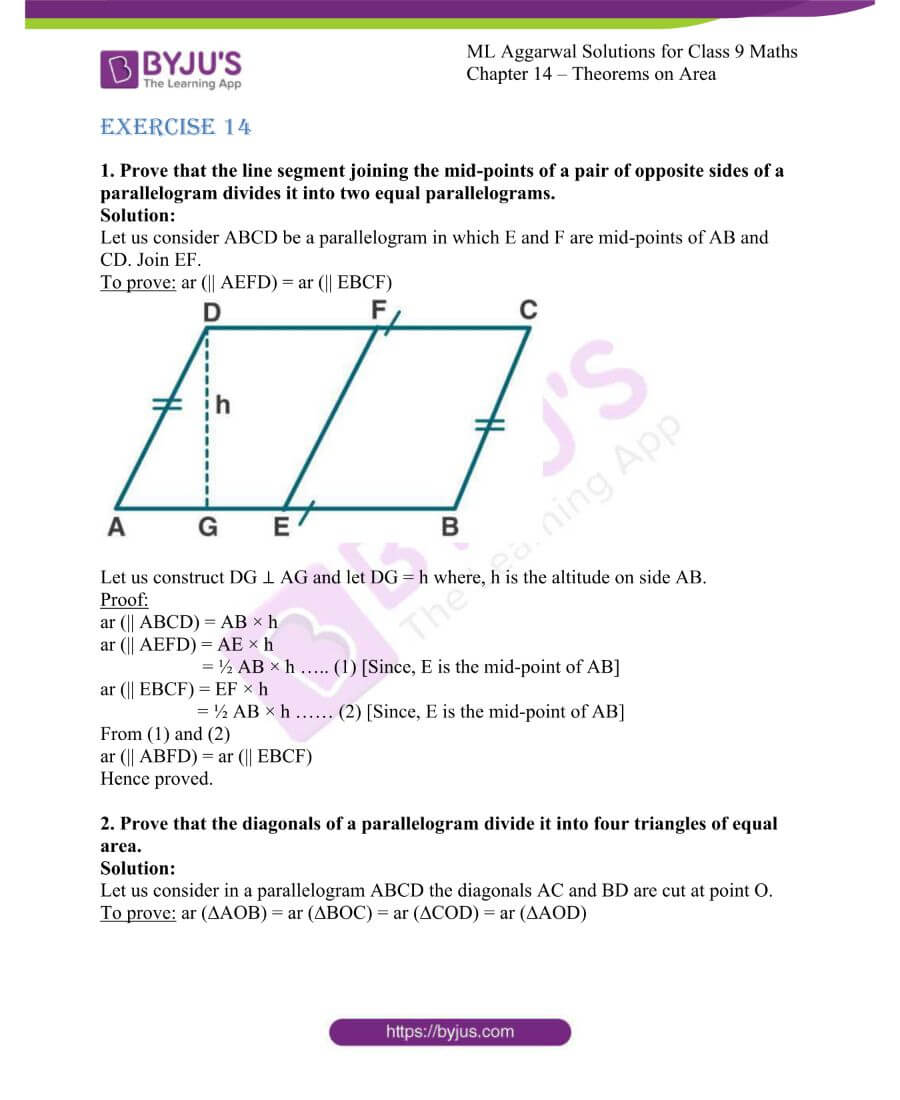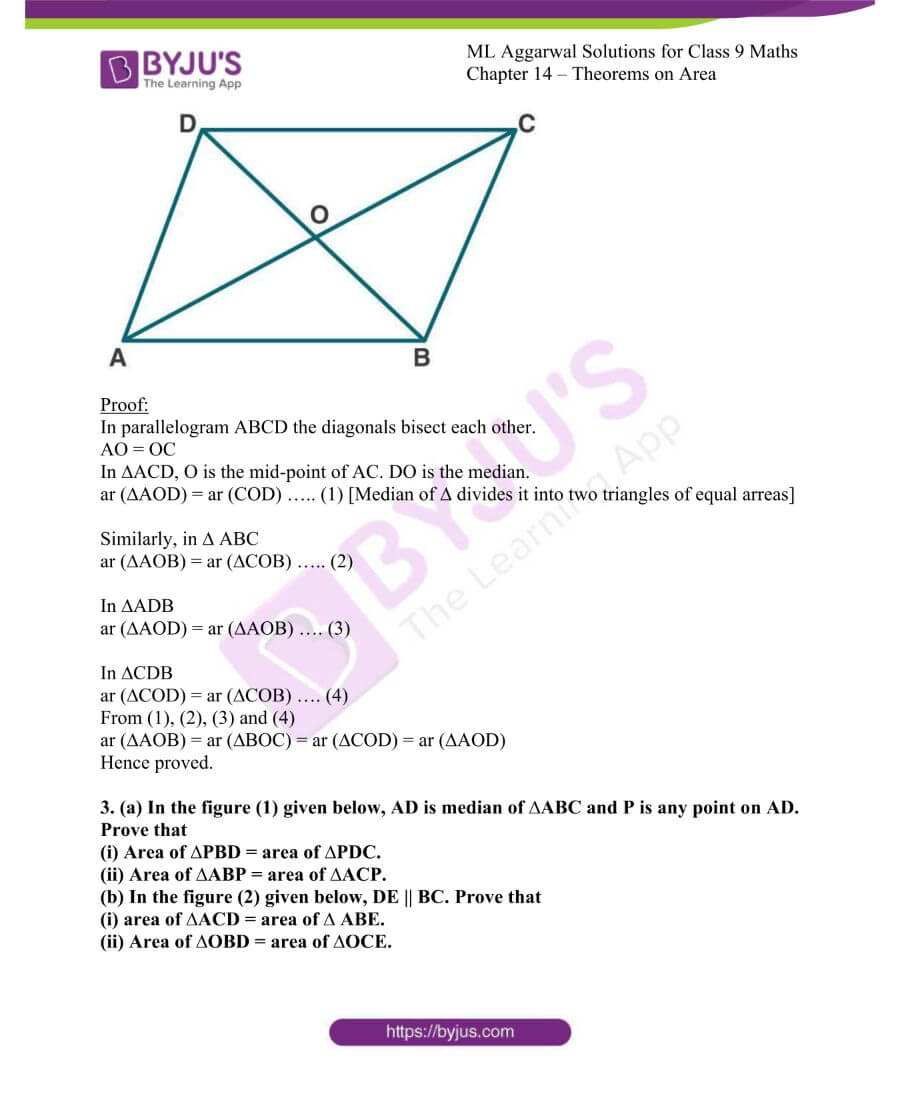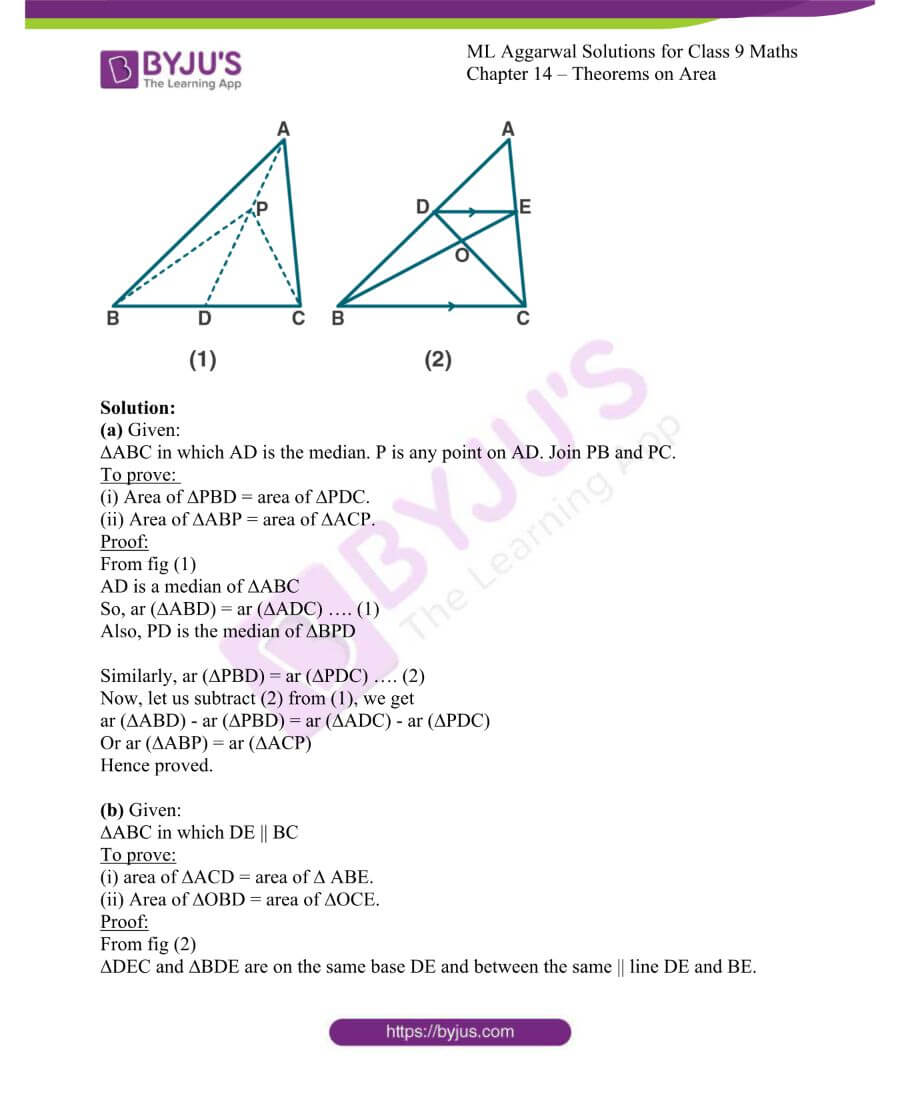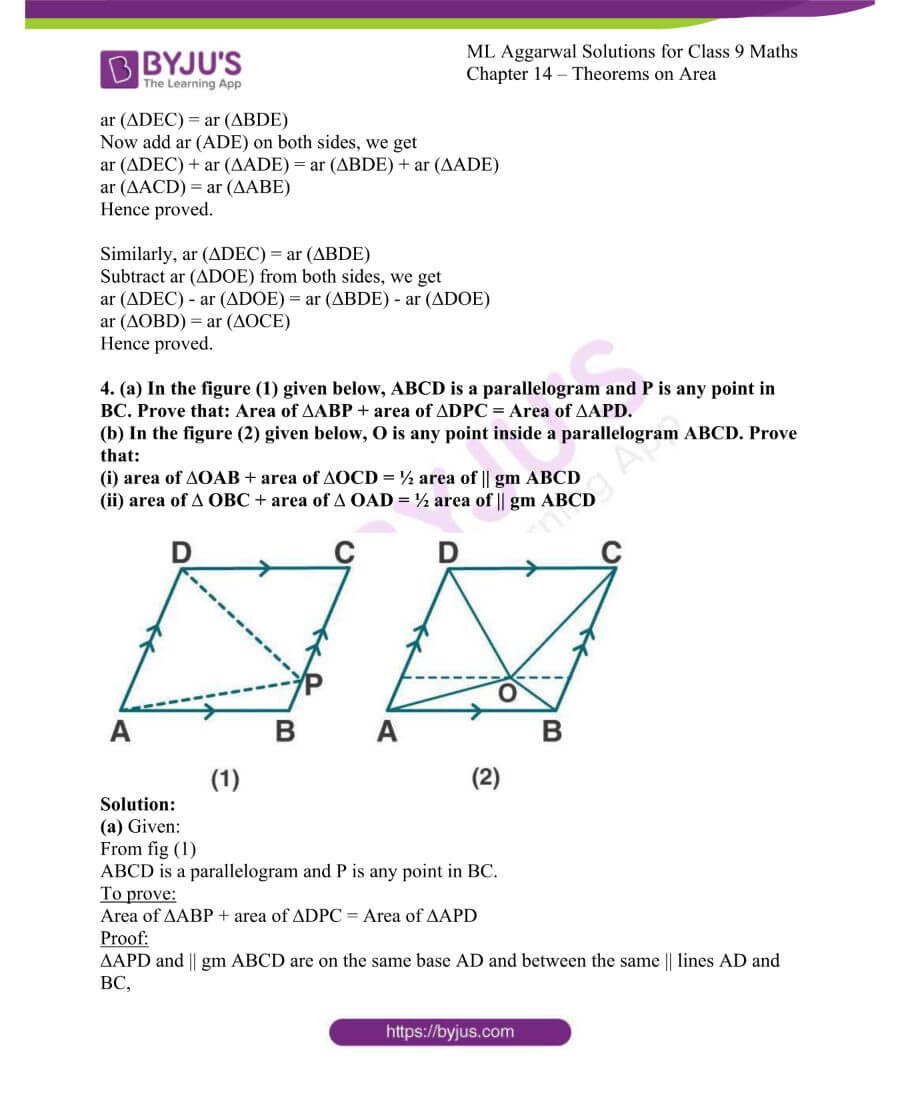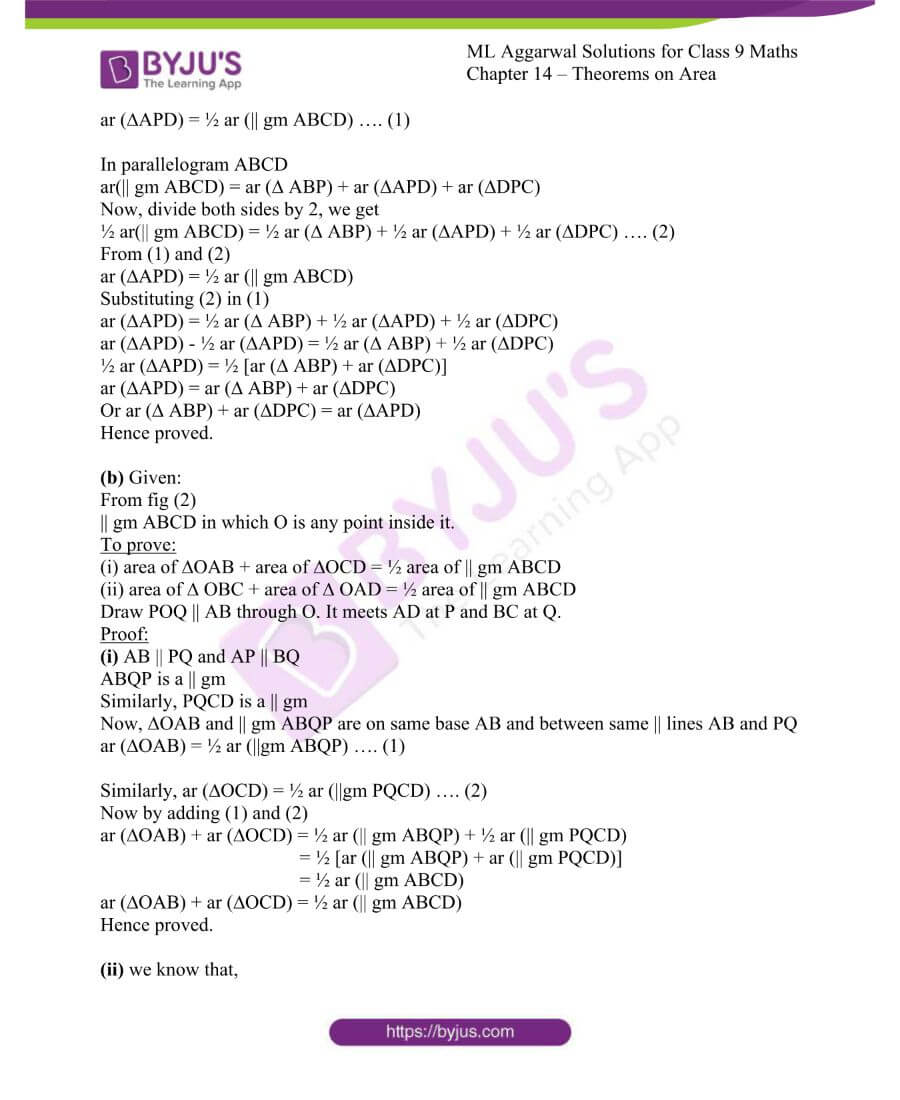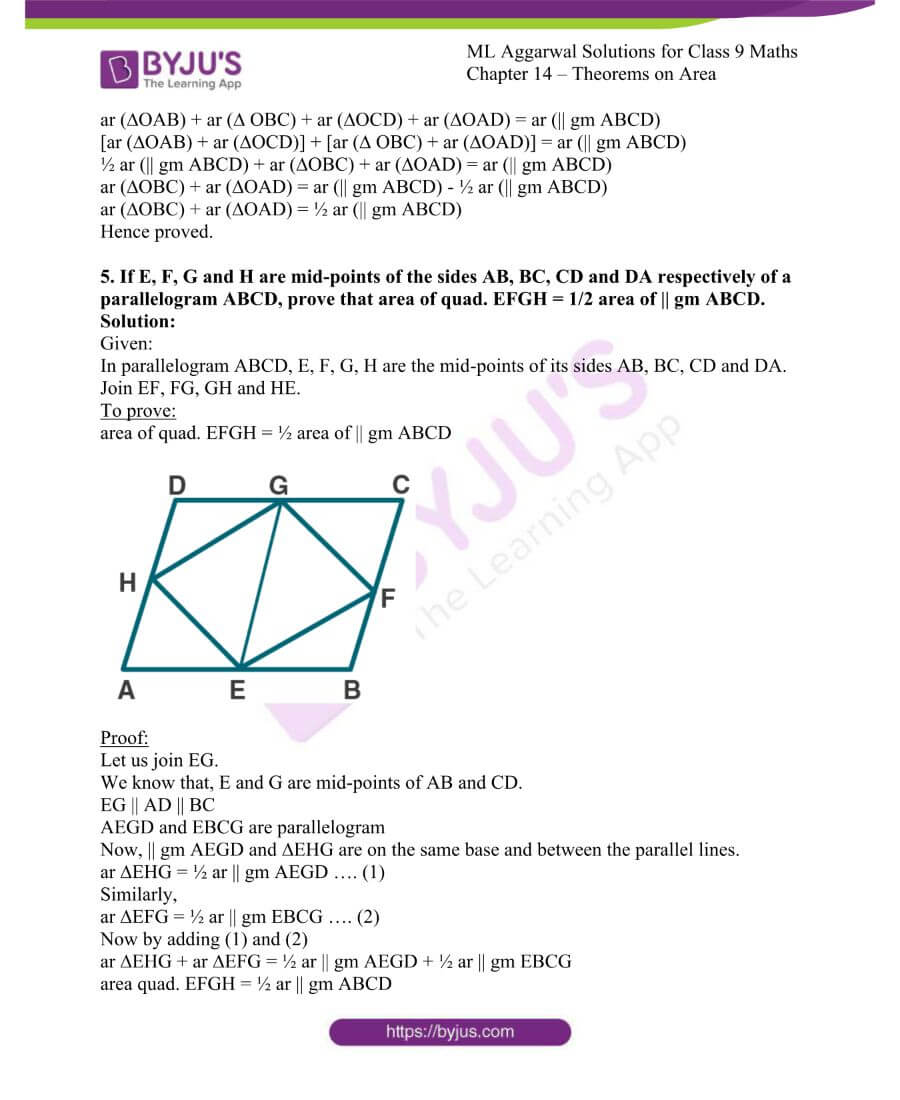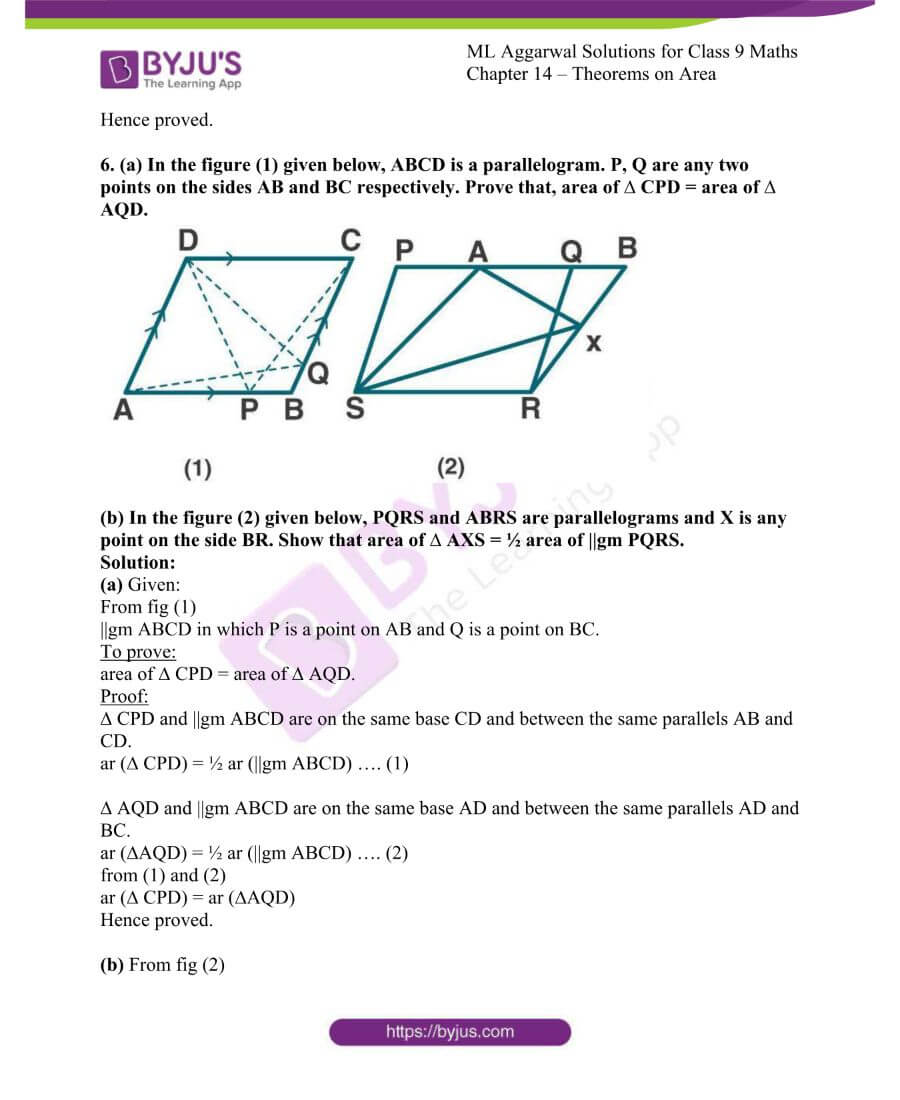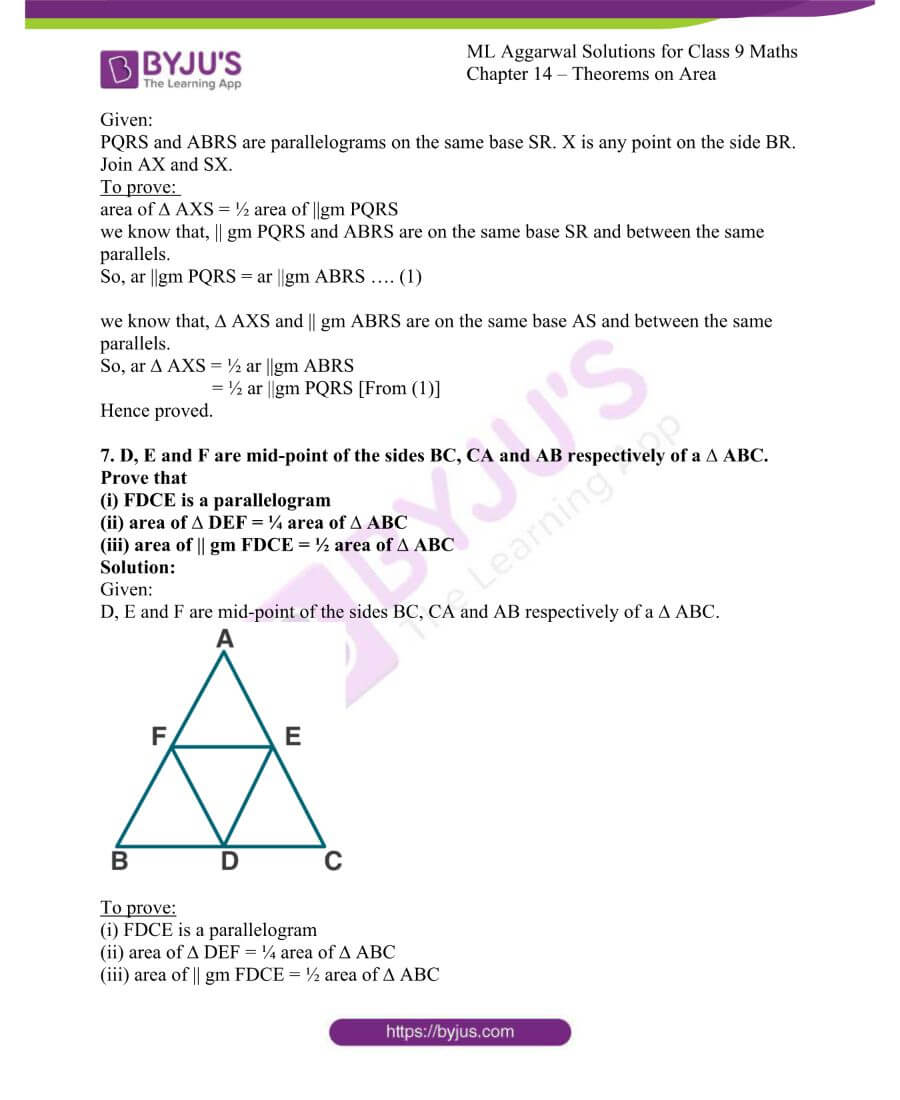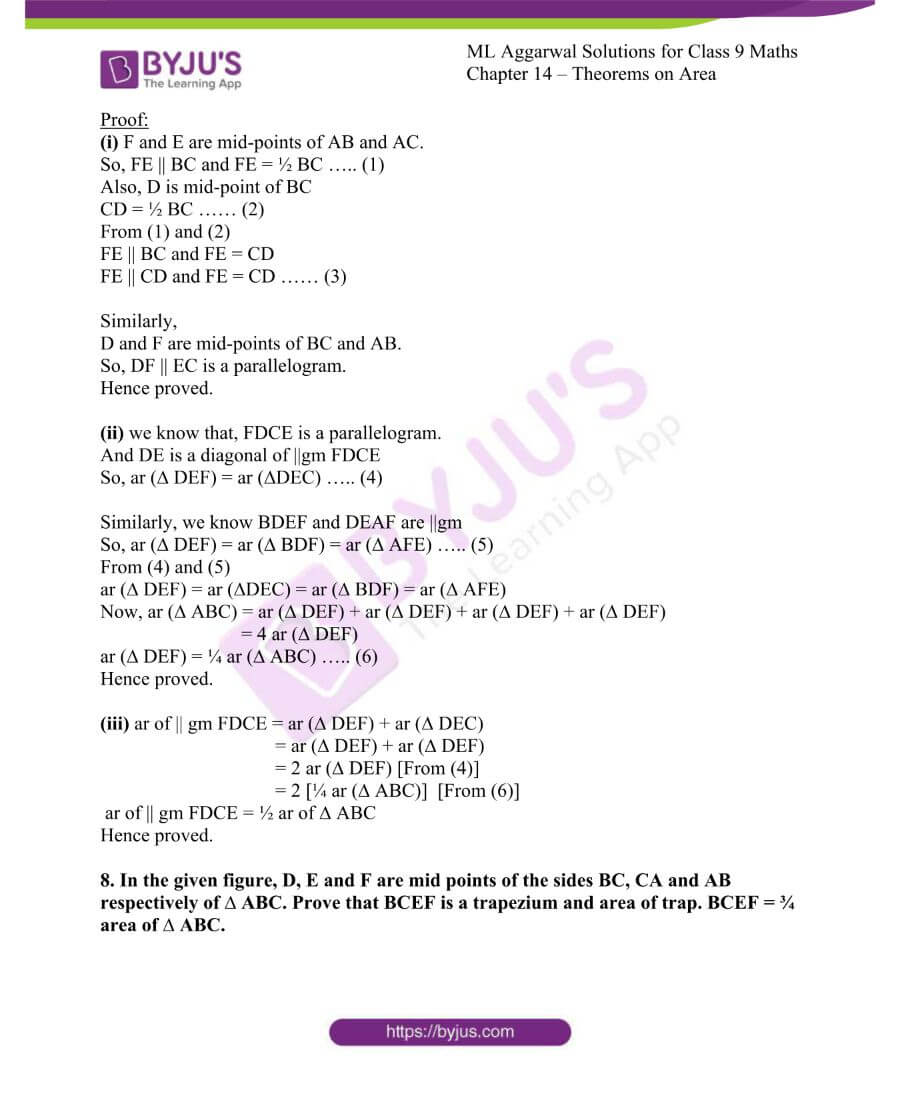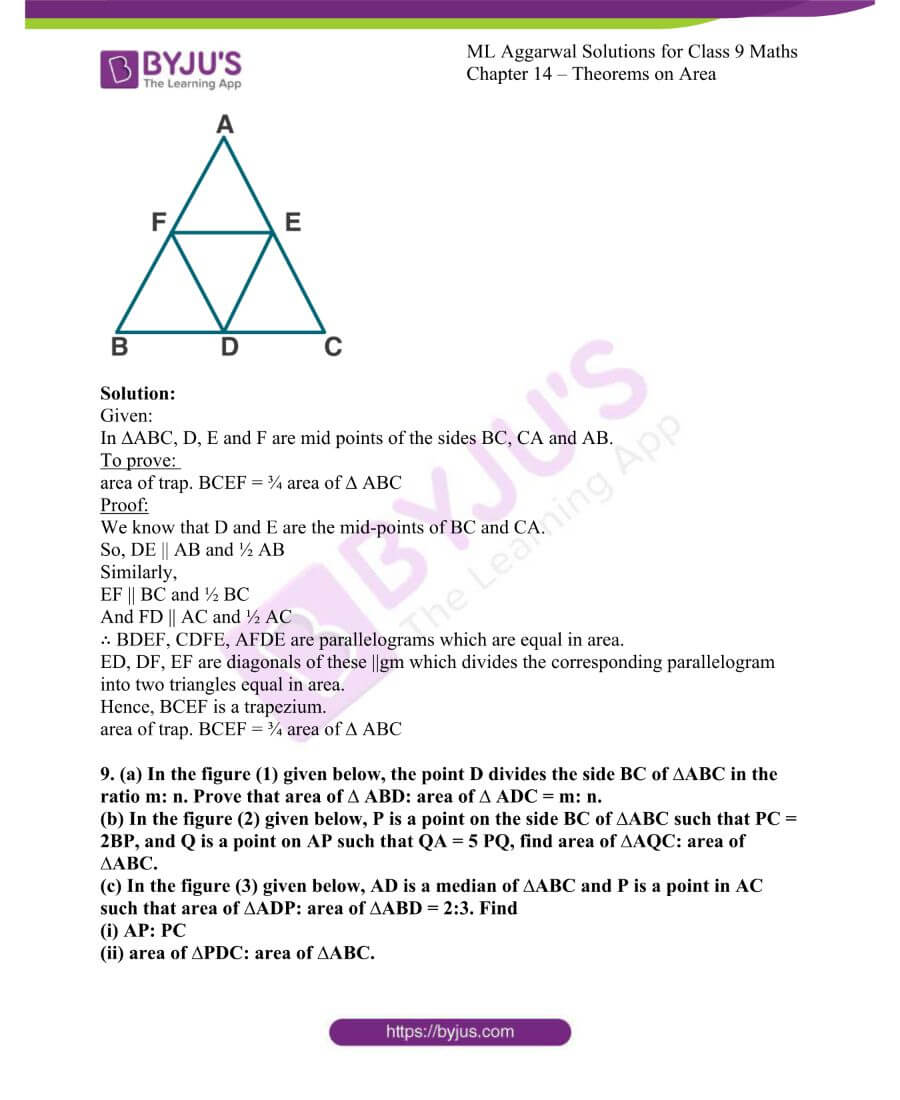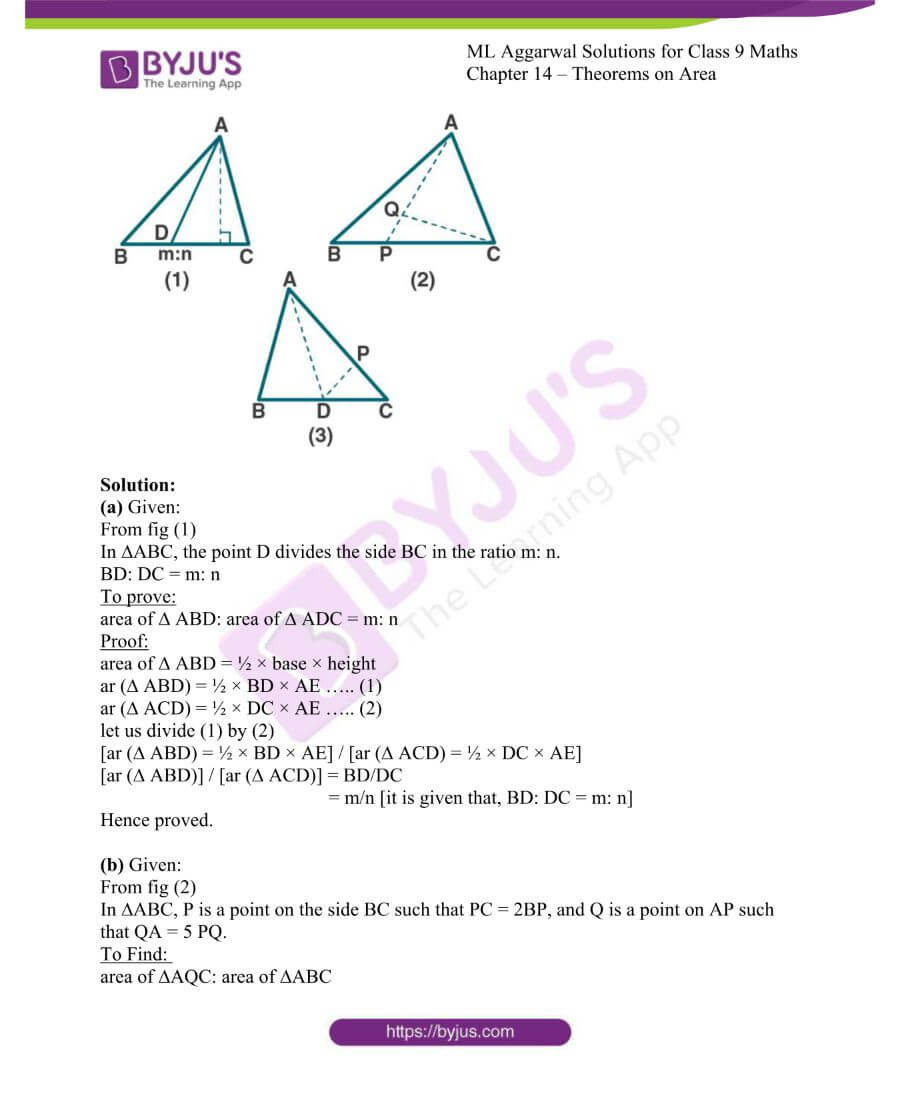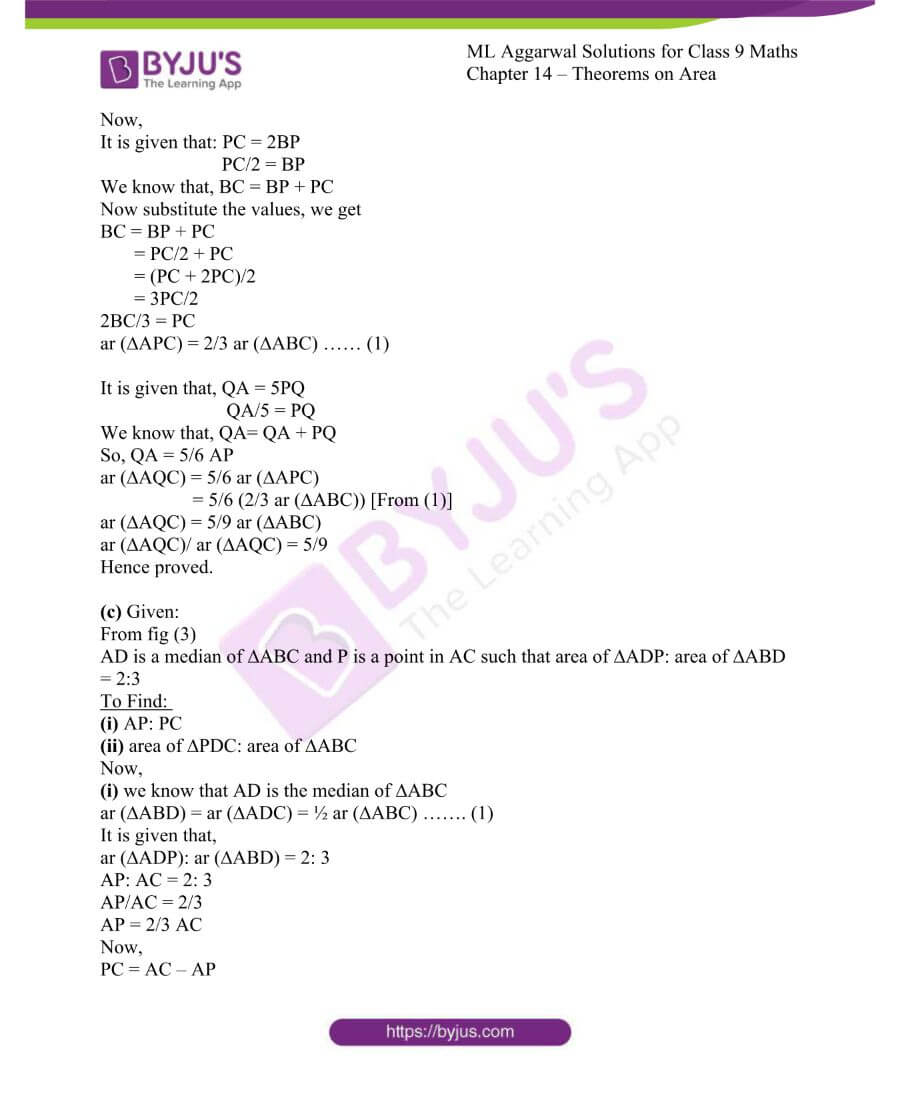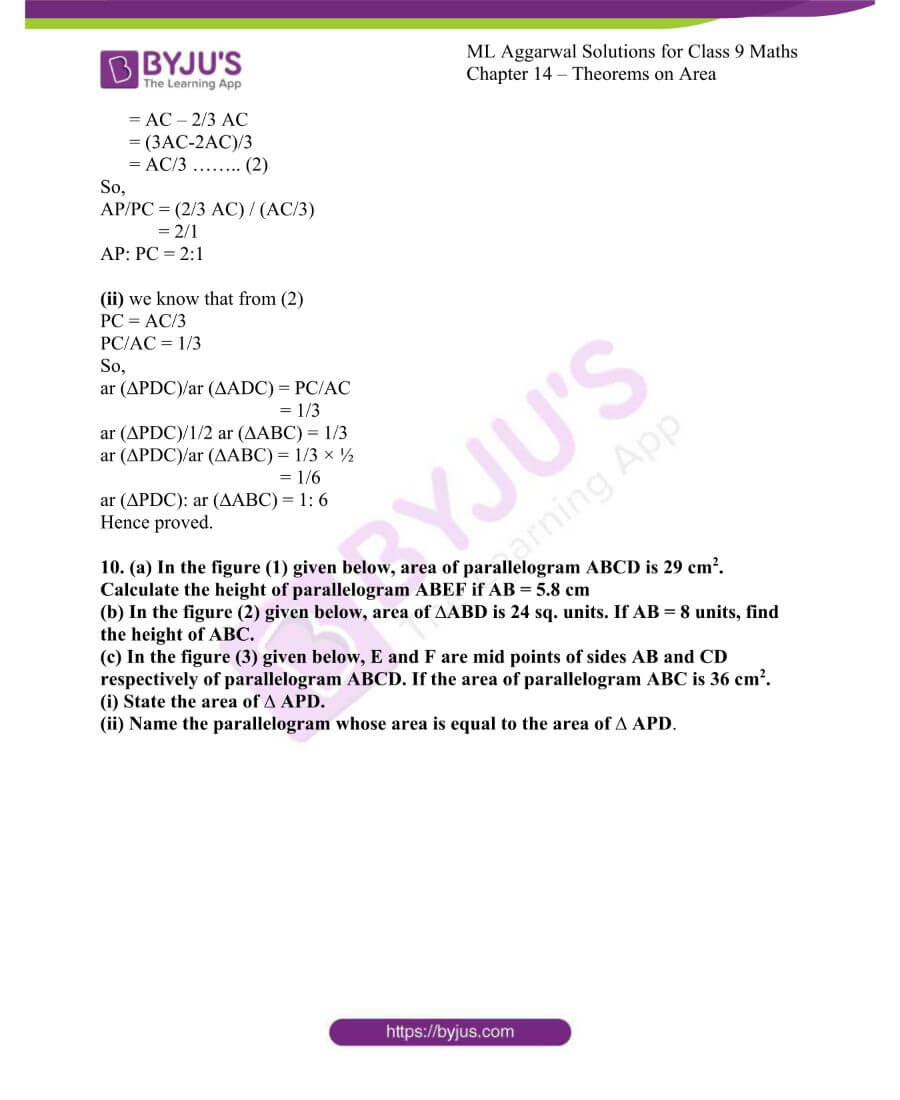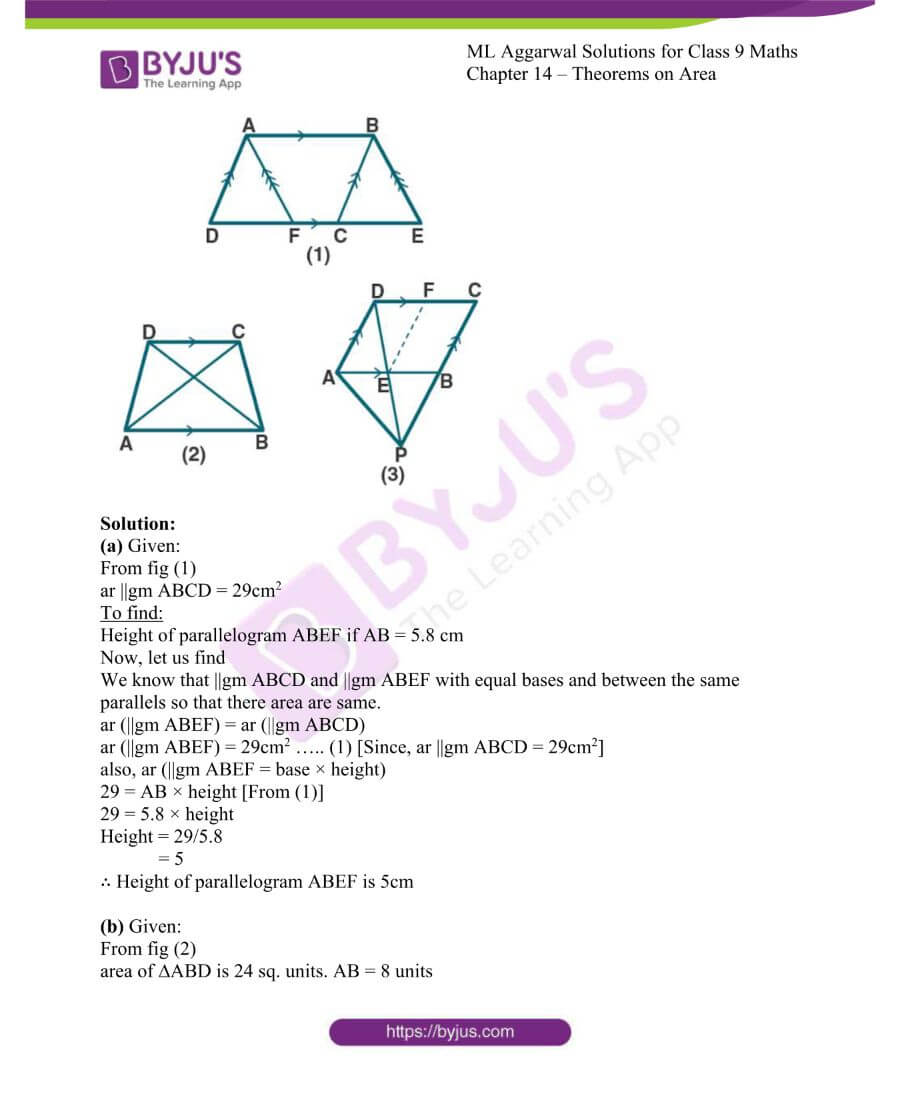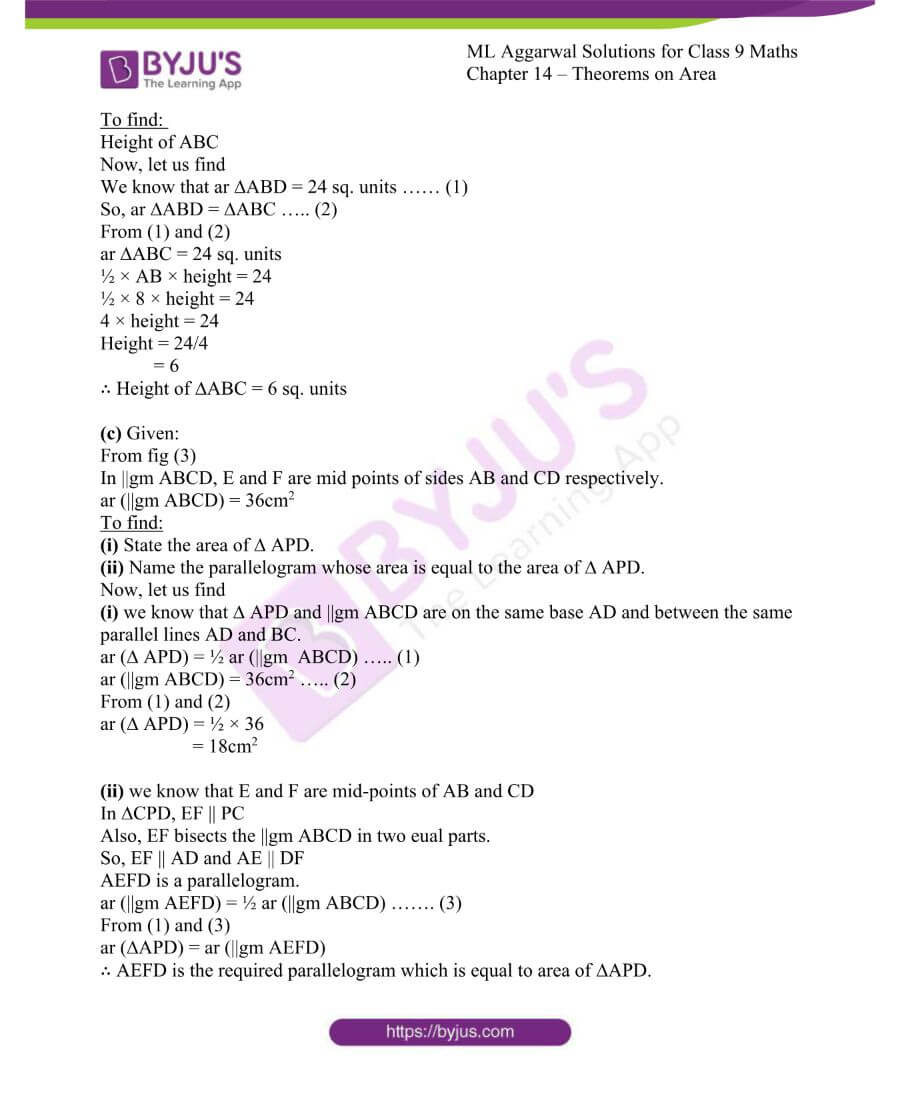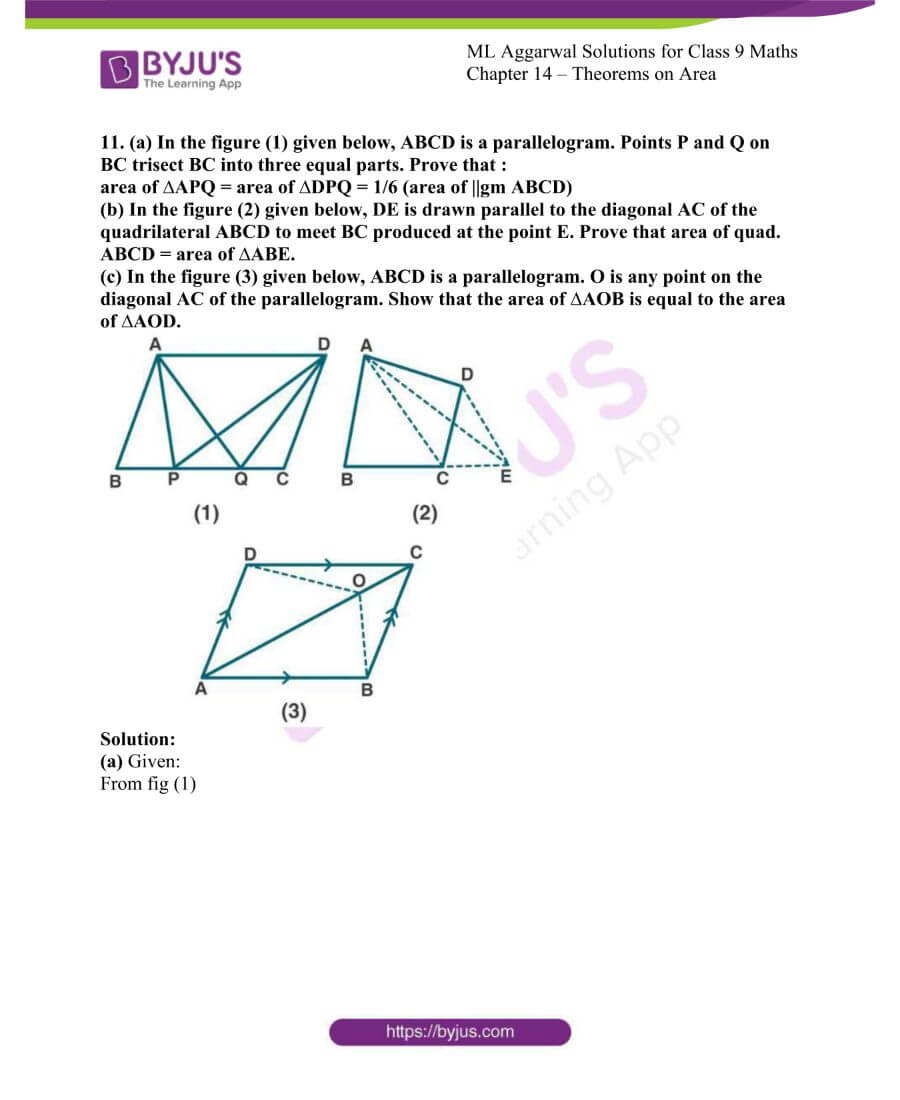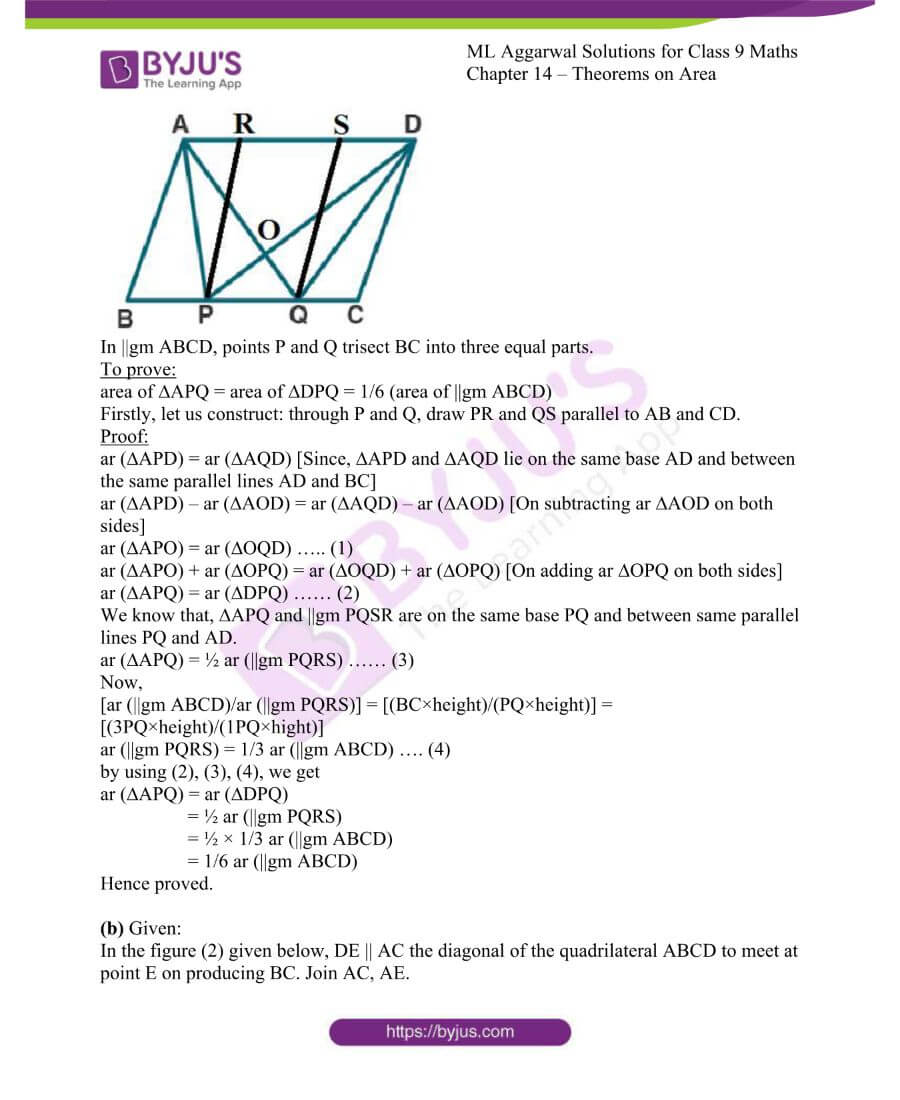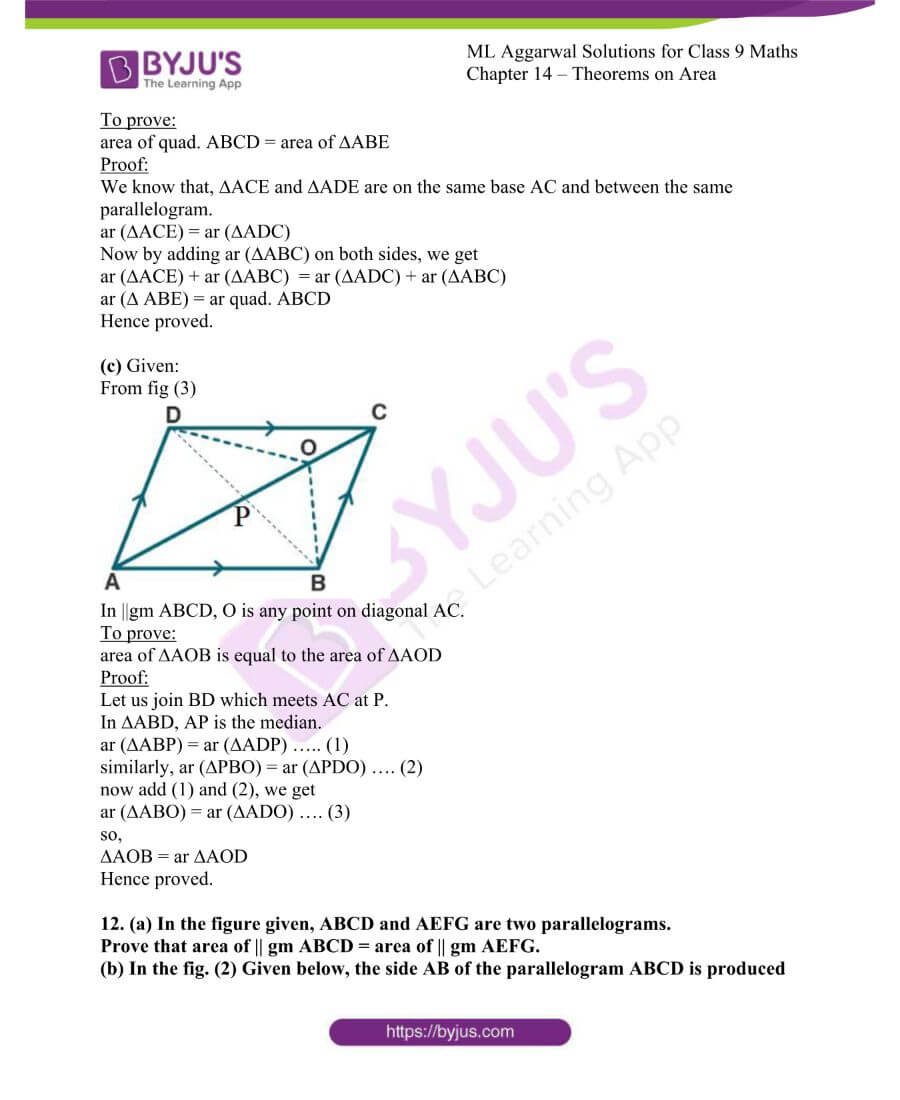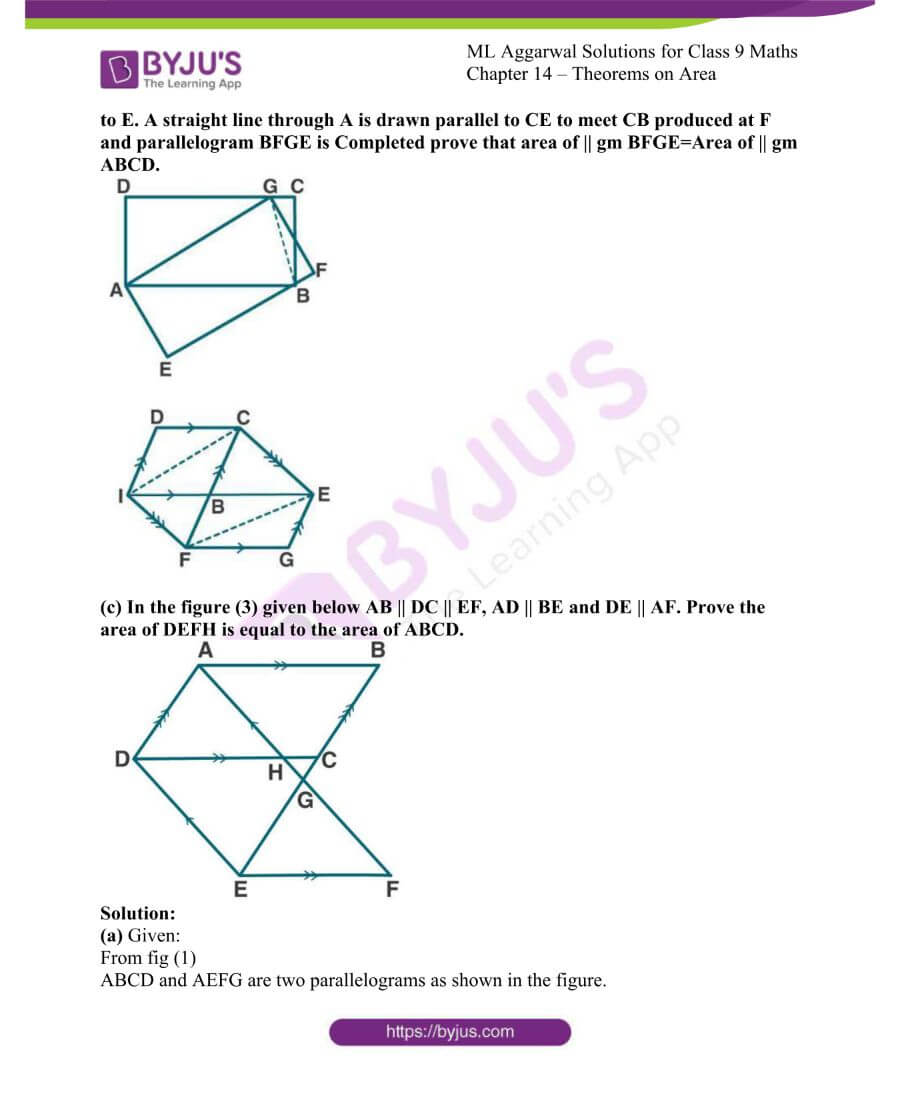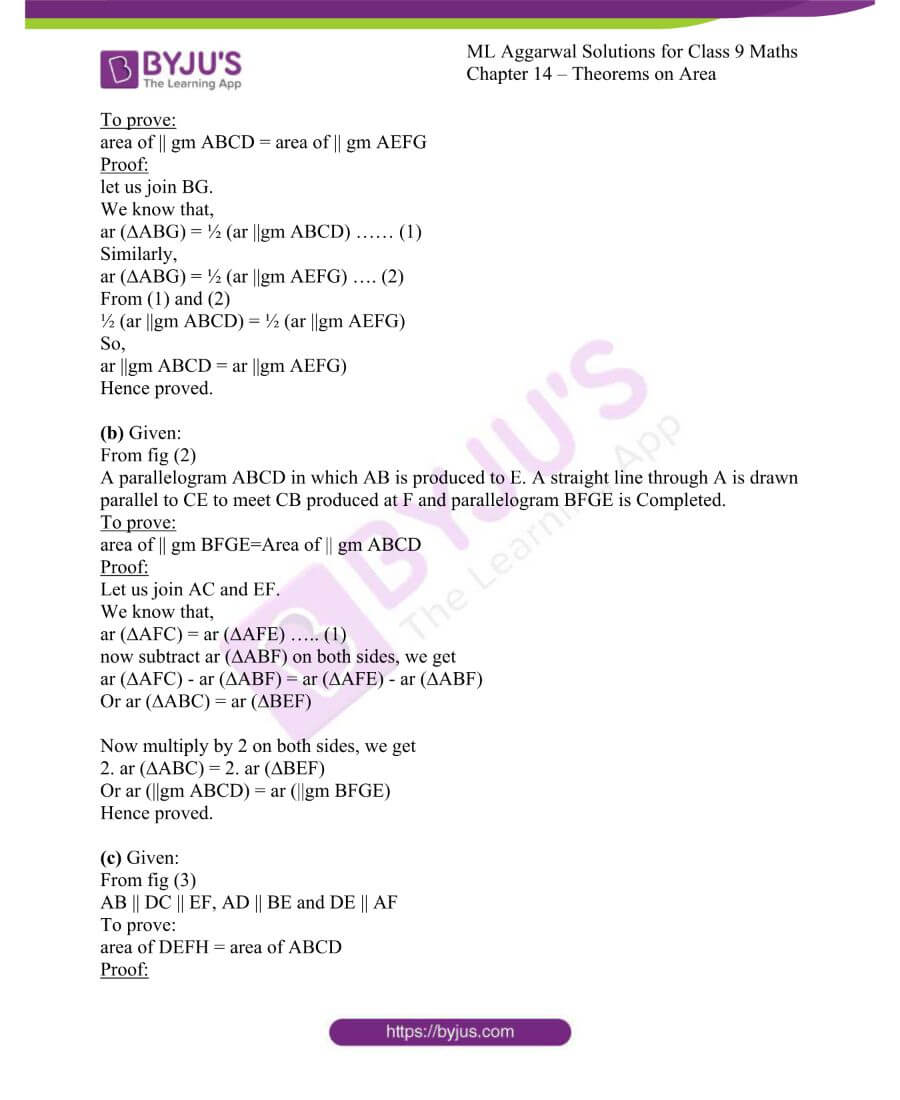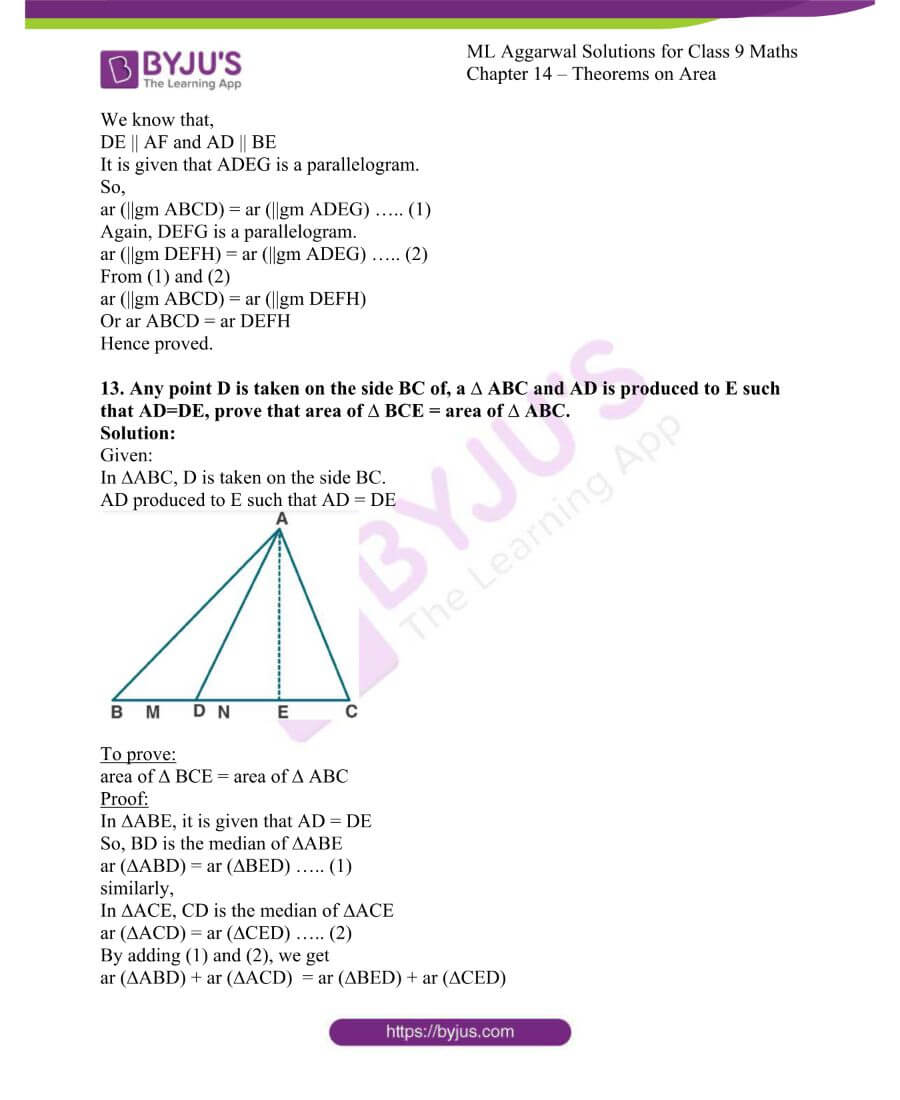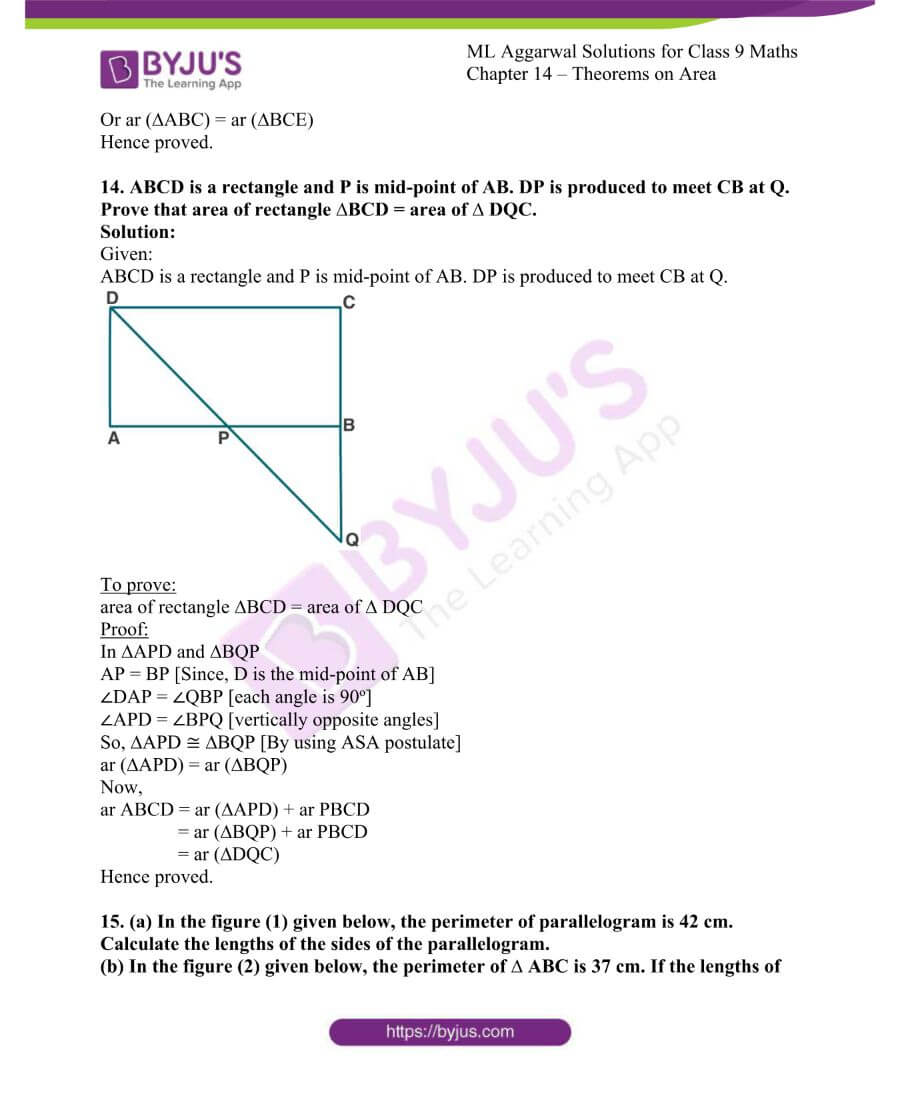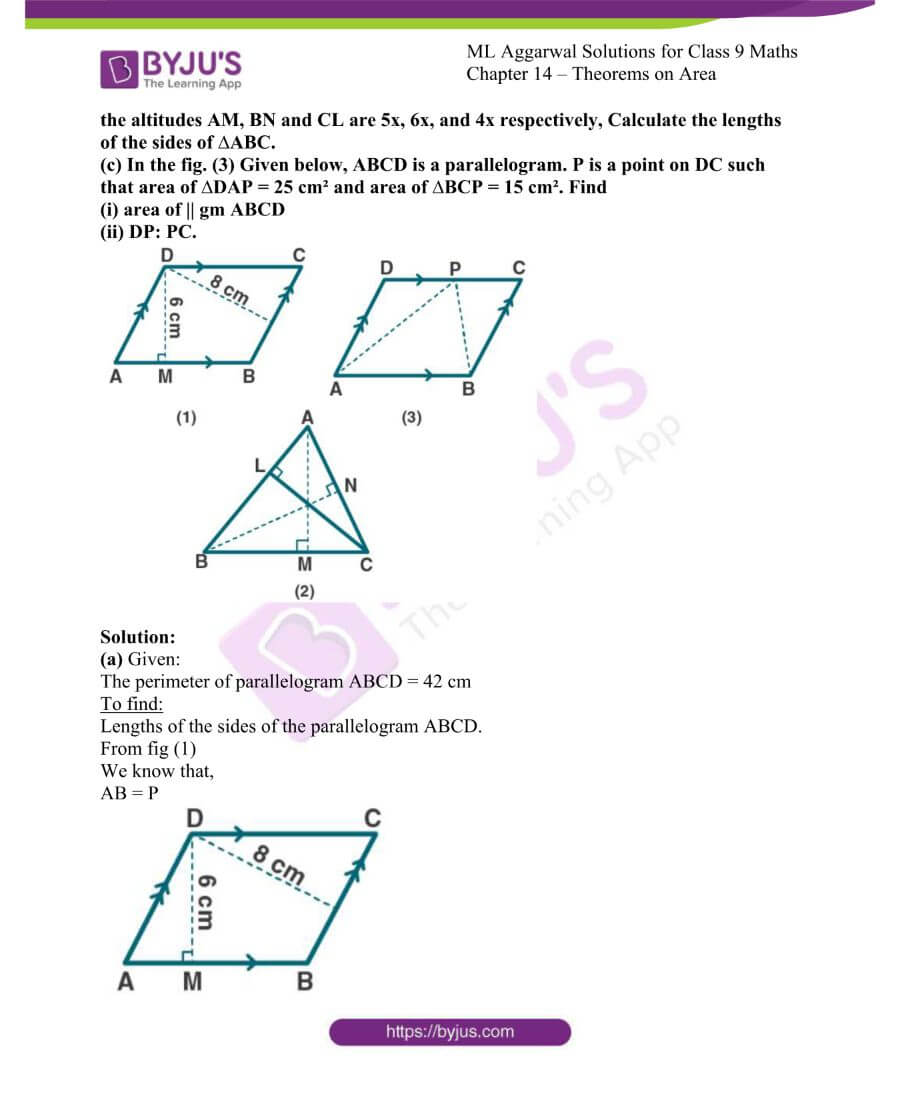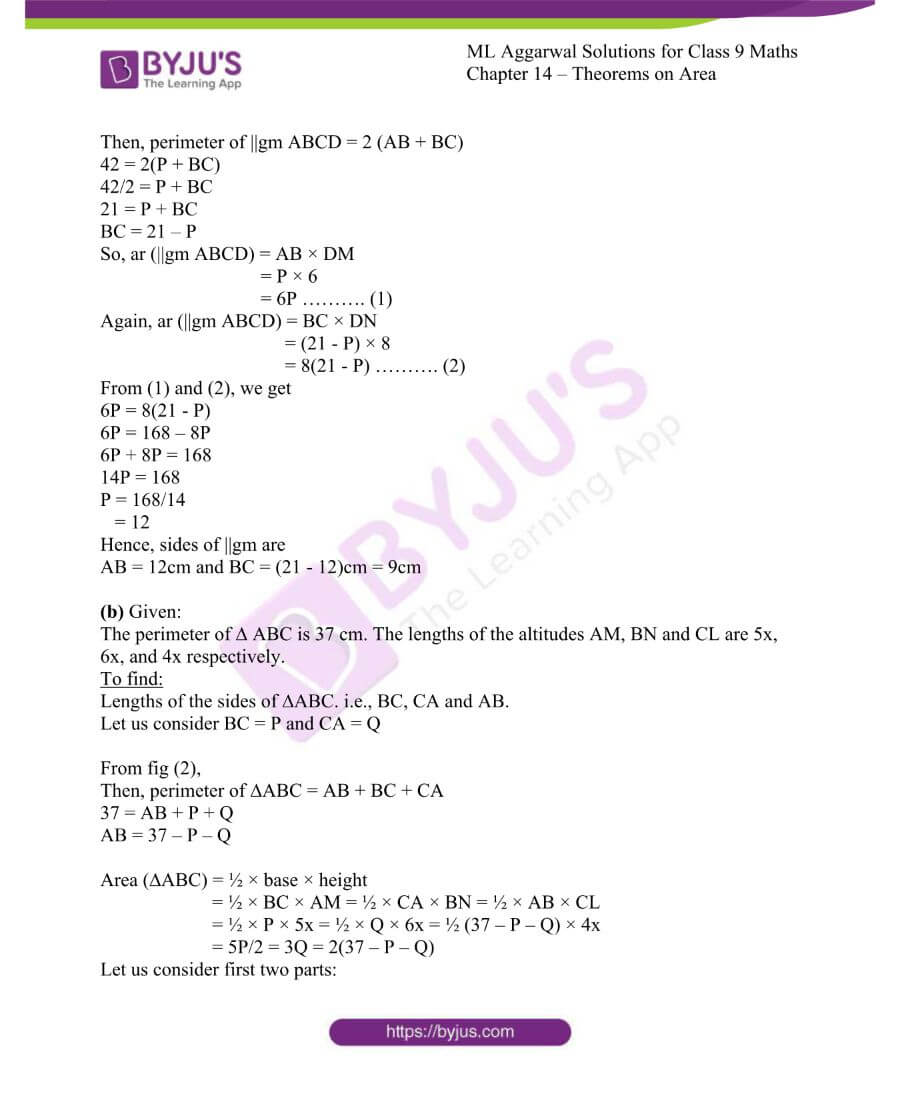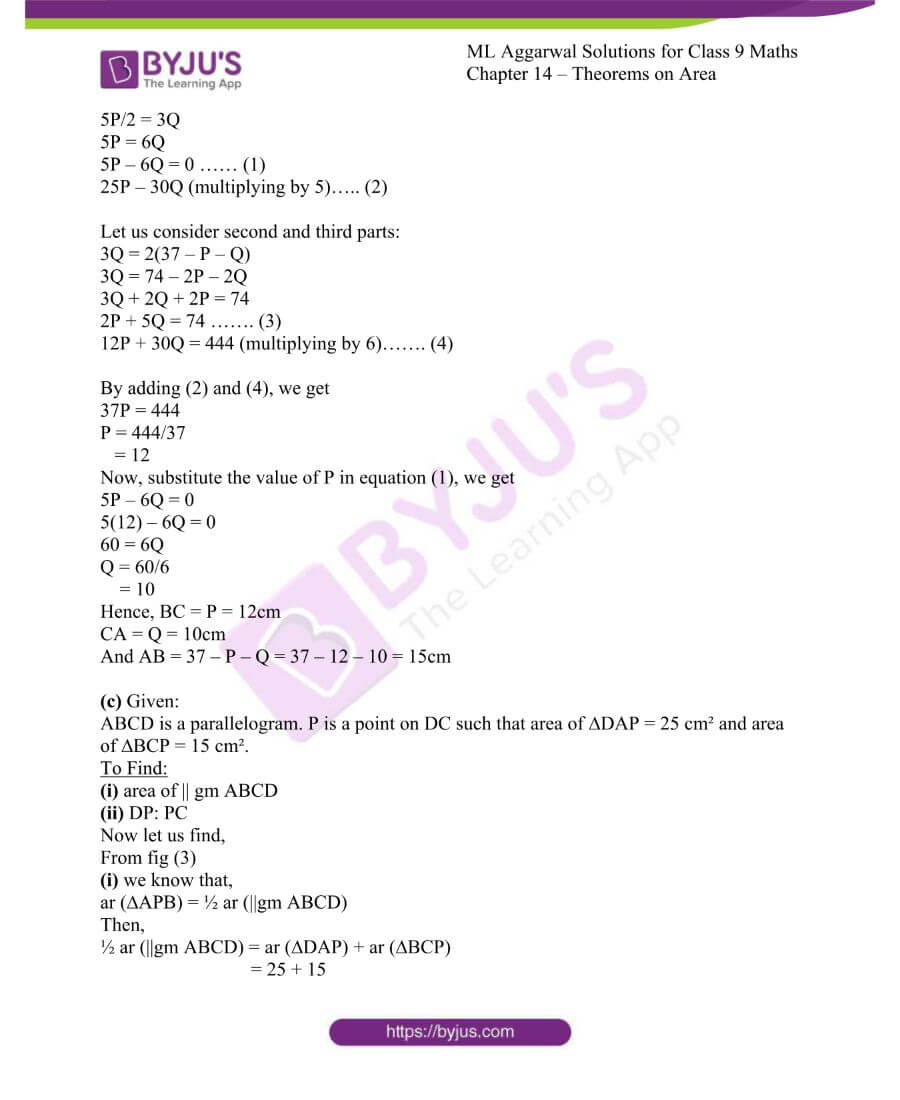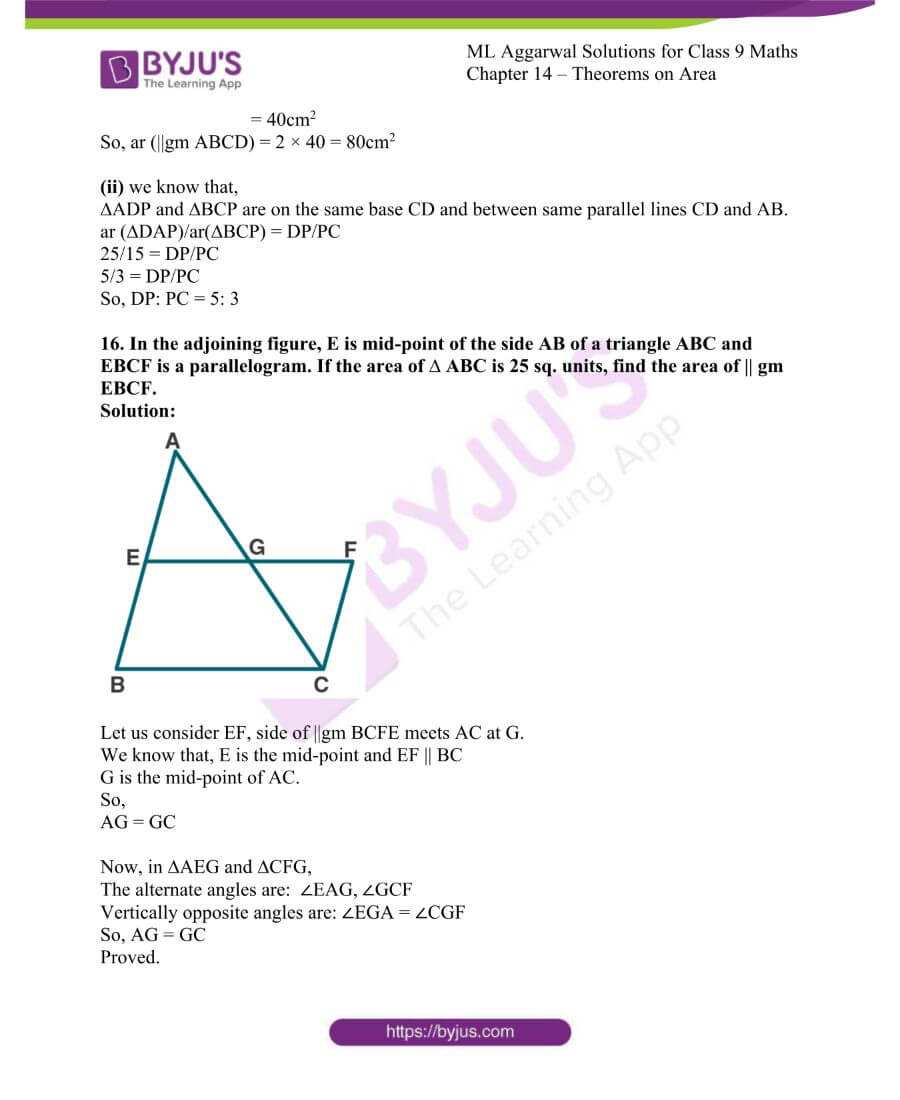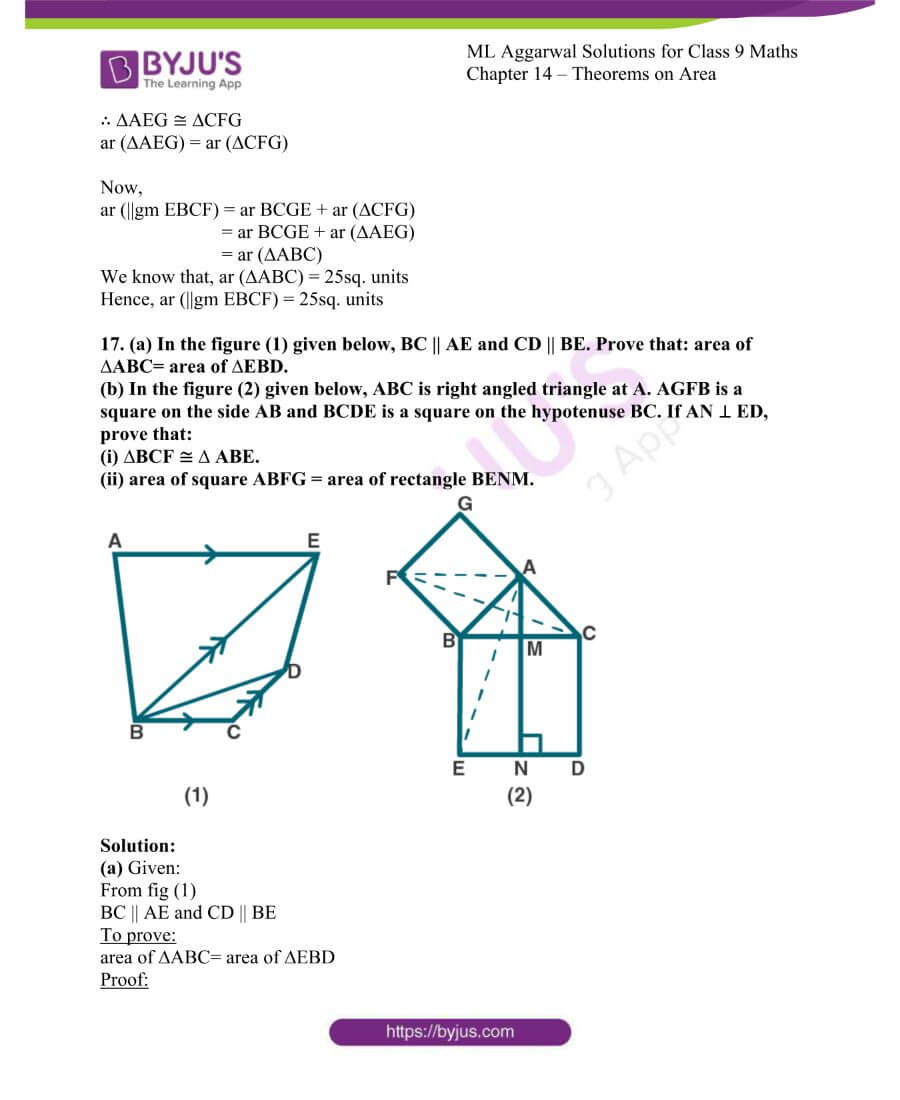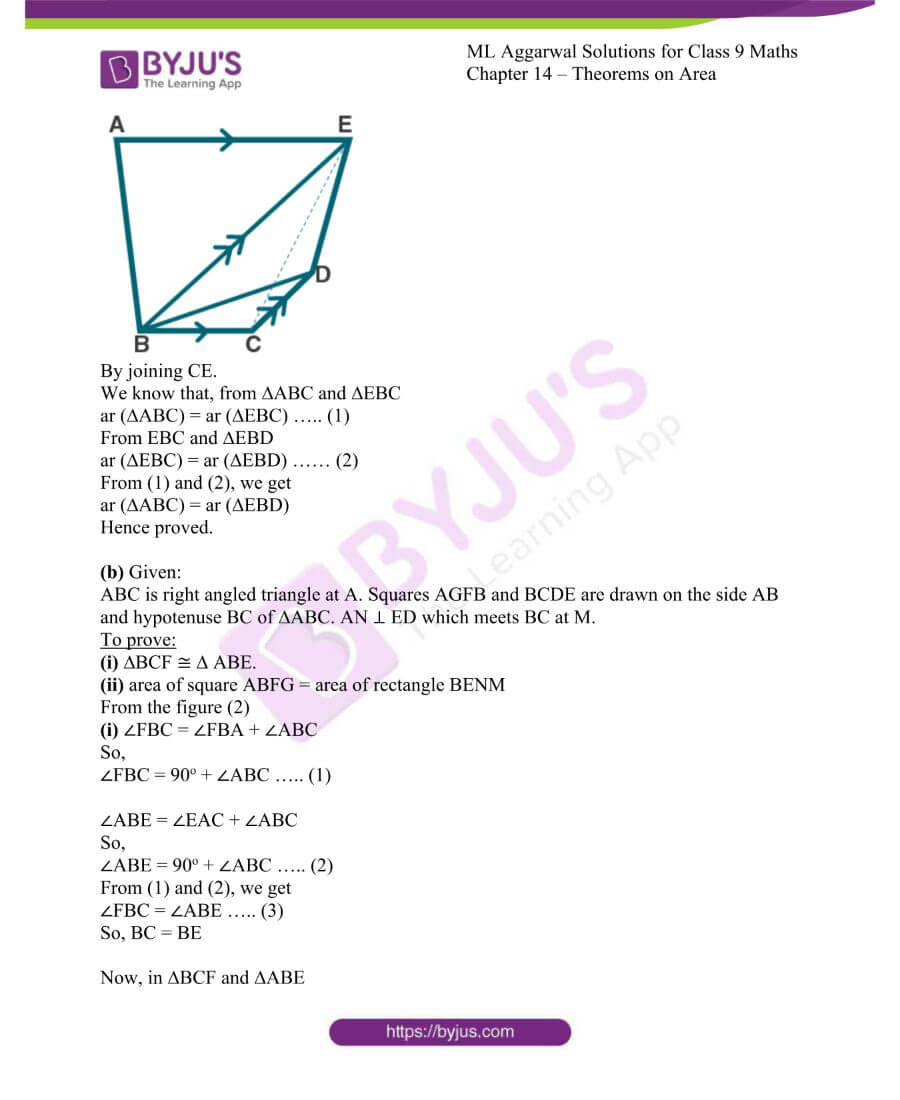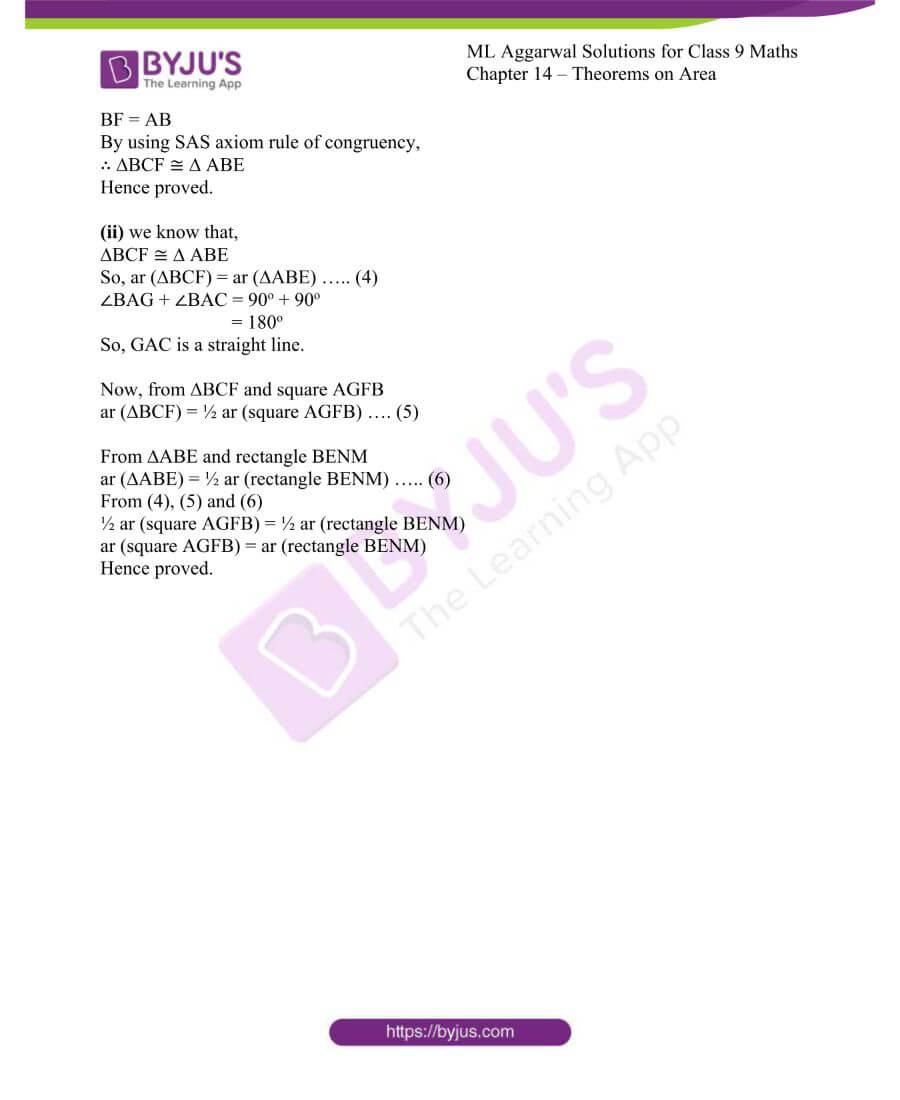### Access answers to ML Aggarwal Solutions for Class 9 Maths Chapter 14 – Theorems on Area.

EXERCISE 14

1. Prove that the line segment joining the mid-points of a pair of opposite sides of a parallelogram divides it into two equal parallelograms.

Solution:

Let us consider ABCD be a parallelogram in which E and F are mid-points of AB and CD. Join EF.

To prove: ar (|| AEFD) = ar (|| EBCF)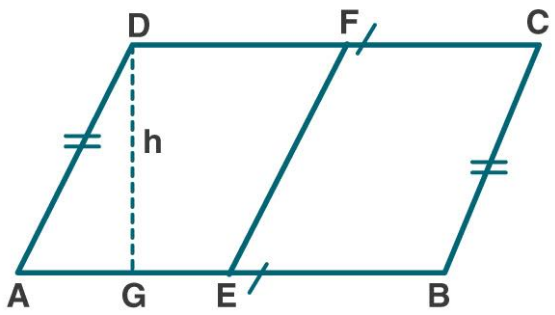Let us construct DG ⊥ AG and let DG = h where, h is the altitude on side AB.

Proof:

ar (|| ABCD) = AB × h

ar (|| AEFD) = AE × h

= ½ AB × h ….. (1) [Since, E is the mid-point of AB]

ar (|| EBCF) = EF × h

= ½ AB × h …… (2) [Since, E is the mid-point of AB]

From (1) and (2)

ar (|| ABFD) = ar (|| EBCF)

Hence proved.

2. Prove that the diagonals of a parallelogram divide it into four triangles of equal area.

Solution:

Let us consider in a parallelogram ABCD the diagonals AC and BD are cut at point O.

To prove: ar (∆AOB) = ar (∆BOC) = ar (∆COD) = ar (∆AOD)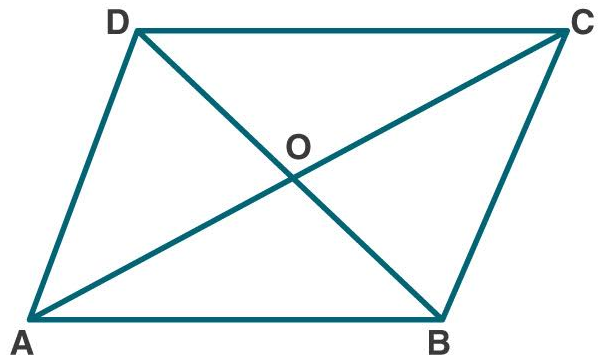Proof:

In parallelogram ABCD the diagonals bisect each other.

AO = OC

In ∆ACD, O is the mid-point of AC. DO is the median.

ar (∆AOD) = ar (COD) ….. (1) [Median of ∆ divides it into two triangles of equal arreas]

Similarly, in ∆ ABC

ar (∆AOB) = ar (∆COB) ….. (2)

ar (∆AOD) = ar (∆AOB) …. (3)

In ∆CDB

ar (∆COD) = ar (∆COB) …. (4)

From (1), (2), (3) and (4)

ar (∆AOB) = ar (∆BOC) = ar (∆COD) = ar (∆AOD)

Hence proved.

3. (a) In the figure (1) given below, AD is median of ∆ABC and P is any point on AD. Prove that

(i) Area of ∆PBD = area of ∆PDC.

(ii) Area of ∆ABP = area of ∆ACP.

(b) In the figure (2) given below, DE || BC. Prove that

(i) area of ∆ACD = area of ∆ ABE.

(ii) Area of ∆OBD = area of ∆OCE.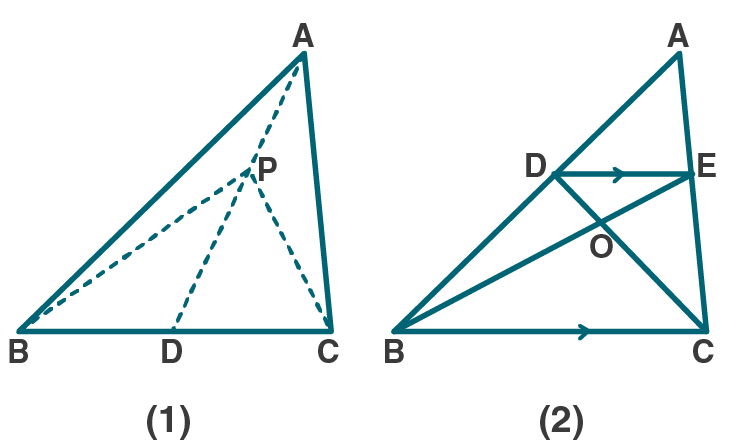Solution:

(a) Given:

∆ABC in which AD is the median. P is any point on AD. Join PB and PC.

To prove:

(i) Area of ∆PBD = area of ∆PDC.

(ii) Area of ∆ABP = area of ∆ACP.

Proof:

From fig (1)

AD is a median of ∆ABC

So, ar (∆ABD) = ar (∆ADC) …. (1)

Also, PD is the median of ∆BPD

Similarly, ar (∆PBD) = ar (∆PDC) …. (2)

Now, let us subtract (2) from (1), we get

ar (∆ABD) – ar (∆PBD) = ar (∆ADC) – ar (∆PDC)

Or ar (∆ABP) = ar (∆ACP)

Hence proved.

(b) Given:

∆ABC in which DE || BC

To prove:

(i) area of ∆ACD = area of ∆ ABE.

(ii) Area of ∆OBD = area of ∆OCE.

Proof:

From fig (2)

∆DEC and ∆BDE are on the same base DE and between the same || line DE and BE.

ar (∆DEC) = ar (∆BDE)

ar (∆ACD) = ar (∆ABE)

Hence proved.

Similarly, ar (∆DEC) = ar (∆BDE)

Subtract ar (∆DOE) from both sides, we get

ar (∆DEC) – ar (∆DOE) = ar (∆BDE) – ar (∆DOE)

ar (∆OBD) = ar (∆OCE)

Hence proved.

4. (a) In the figure (1) given below, ABCD is a parallelogram and P is any point in BC. Prove that: Area of ∆ABP + area of ∆DPC = Area of ∆APD.

(b) In the figure (2) given below, O is any point inside a parallelogram ABCD. Prove that:

(i) area of ∆OAB + area of ∆OCD = ½ area of || gm ABCD

(ii) area of ∆ OBC + area of ∆ OAD = ½ area of || gm ABCD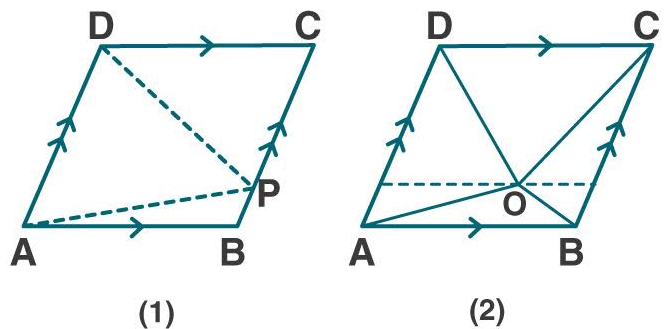Solution:

(a) Given:

From fig (1)

ABCD is a parallelogram and P is any point in BC.

To prove:

Area of ∆ABP + area of ∆DPC = Area of ∆APD

Proof:

∆APD and || gm ABCD are on the same base AD and between the same || lines AD and BC,

ar (∆APD) = ½ ar (|| gm ABCD) …. (1)

In parallelogram ABCD

ar(|| gm ABCD) = ar (∆ ABP) + ar (∆APD) + ar (∆DPC)

Now, divide both sides by 2, we get

½ ar(|| gm ABCD) = ½ ar (∆ ABP) + ½ ar (∆APD) + ½ ar (∆DPC) …. (2)

From (1) and (2)

ar (∆APD) = ½ ar (|| gm ABCD)

Substituting (2) in (1)

ar (∆APD) = ½ ar (∆ ABP) + ½ ar (∆APD) + ½ ar (∆DPC)

ar (∆APD) – ½ ar (∆APD) = ½ ar (∆ ABP) + ½ ar (∆DPC)

½ ar (∆APD) = ½ [ar (∆ ABP) + ar (∆DPC)]

ar (∆APD) = ar (∆ ABP) + ar (∆DPC)

Or ar (∆ ABP) + ar (∆DPC) = ar (∆APD)

Hence proved.

(b) Given:

From fig (2)

|| gm ABCD in which O is any point inside it.

To prove:

(i) area of ∆OAB + area of ∆OCD = ½ area of || gm ABCD

(ii) area of ∆ OBC + area of ∆ OAD = ½ area of || gm ABCD

Draw POQ || AB through O. It meets AD at P and BC at Q.

Proof:

(i) AB || PQ and AP || BQ

ABQP is a || gm

Similarly, PQCD is a || gm

Now, ∆OAB and || gm ABQP are on same base AB and between same || lines AB and PQ

ar (∆OAB) = ½ ar (||gm ABQP) …. (1)

Similarly, ar (∆OCD) = ½ ar (||gm PQCD) …. (2)

Now by adding (1) and (2)

ar (∆OAB) + ar (∆OCD) = ½ ar (|| gm ABQP) + ½ ar (|| gm PQCD)

= ½ [ar (|| gm ABQP) + ar (|| gm PQCD)]

= ½ ar (|| gm ABCD)

ar (∆OAB) + ar (∆OCD) = ½ ar (|| gm ABCD)

Hence proved.

(ii) we know that,

ar (∆OAB) + ar (∆ OBC) + ar (∆OCD) + ar (∆OAD) = ar (|| gm ABCD)

[ar (∆OAB) + ar (∆OCD)] + [ar (∆ OBC) + ar (∆OAD)] = ar (|| gm ABCD)

½ ar (|| gm ABCD) + ar (∆OBC) + ar (∆OAD) = ar (|| gm ABCD)

ar (∆OBC) + ar (∆OAD) = ar (|| gm ABCD) – ½ ar (|| gm ABCD)

ar (∆OBC) + ar (∆OAD) = ½ ar (|| gm ABCD)

Hence proved.

5. If E, F, G and H are mid-points of the sides AB, BC, CD and DA respectively of a parallelogram ABCD, prove that area of quad. EFGH = 1/2 area of || gm ABCD.

Solution:

Given:

In parallelogram ABCD, E, F, G, H are the mid-points of its sides AB, BC, CD and DA.

Join EF, FG, GH and HE.

To prove:

area of quad. EFGH = ½ area of || gm ABCD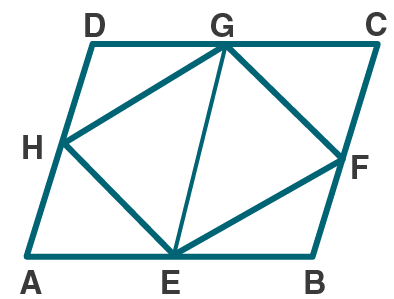Proof:

Let us join EG.

We know that, E and G are mid-points of AB and CD.

AEGD and EBCG are parallelogram

Now, || gm AEGD and ∆EHG are on the same base and between the parallel lines.

ar ∆EHG = ½ ar || gm AEGD …. (1)

Similarly,

ar ∆EFG = ½ ar || gm EBCG …. (2)

Now by adding (1) and (2)

ar ∆EHG + ar ∆EFG = ½ ar || gm AEGD + ½ ar || gm EBCG

area quad. EFGH = ½ ar || gm ABCD

Hence proved.

6. (a) In the figure (1) given below, ABCD is a parallelogram. P, Q are any two points on the sides AB and BC respectively. Prove that, area of ∆ CPD = area of ∆ AQD.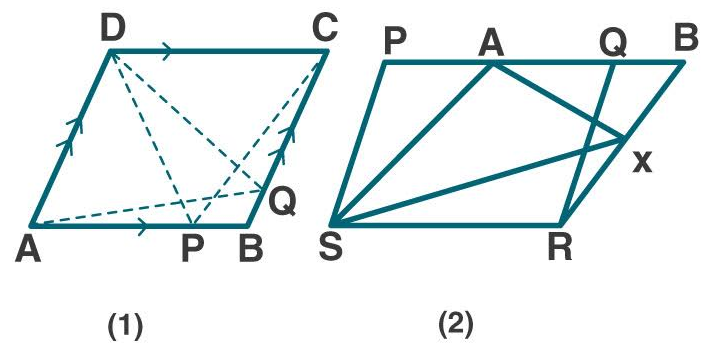(b) In the figure (2) given below, PQRS and ABRS are parallelograms and X is any point on the side BR. Show that area of ∆ AXS = ½ area of ||gm PQRS.

Solution:

(a) Given:

From fig (1)

||gm ABCD in which P is a point on AB and Q is a point on BC.

To prove:

area of ∆ CPD = area of ∆ AQD.

Proof:

∆ CPD and ||gm ABCD are on the same base CD and between the same parallels AB and CD.

ar (∆ CPD) = ½ ar (||gm ABCD) …. (1)

∆ AQD and ||gm ABCD are on the same base AD and between the same parallels AD and BC.

ar (∆AQD) = ½ ar (||gm ABCD) …. (2)

from (1) and (2)

ar (∆ CPD) = ar (∆AQD)

Hence proved.

(b) From fig (2)

Given:

PQRS and ABRS are parallelograms on the same base SR. X is any point on the side BR.

Join AX and SX.

To prove:

area of ∆ AXS = ½ area of ||gm PQRS

we know that, || gm PQRS and ABRS are on the same base SR and between the same parallels.

So, ar ||gm PQRS = ar ||gm ABRS …. (1)

we know that, ∆ AXS and || gm ABRS are on the same base AS and between the same parallels.

So, ar ∆ AXS = ½ ar ||gm ABRS

= ½ ar ||gm PQRS [From (1)]

Hence proved.

7. D, E and F are mid-point of the sides BC, CA and AB respectively of a ∆ ABC. Prove that

(i) FDCE is a parallelogram

(ii) area of ∆ DEF = ¼ area of ∆ ABC

(iii) area of || gm FDCE = ½ area of ∆ ABC

Solution:

Given:

D, E and F are mid-point of the sides BC, CA and AB respectively of a ∆ ABC.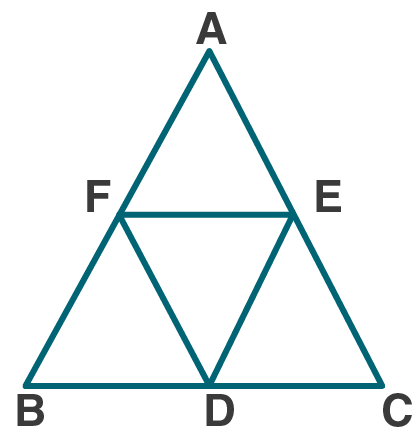To prove:

(i) FDCE is a parallelogram

(ii) area of ∆ DEF = ¼ area of ∆ ABC

(iii) area of || gm FDCE = ½ area of ∆ ABC

Proof:

(i) F and E are mid-points of AB and AC.

So, FE || BC and FE = ½ BC ….. (1)

Also, D is mid-point of BC

CD = ½ BC …… (2)

From (1) and (2)

FE || BC and FE = CD

FE || CD and FE = CD …… (3)

Similarly,

D and F are mid-points of BC and AB.

So, DF || EC is a parallelogram.

Hence proved.

(ii) we know that, FDCE is a parallelogram.

And DE is a diagonal of ||gm FDCE

So, ar (∆ DEF) = ar (∆DEC) ….. (4)

Similarly, we know BDEF and DEAF are ||gm

So, ar (∆ DEF) = ar (∆ BDF) = ar (∆ AFE) ….. (5)

From (4) and (5)

ar (∆ DEF) = ar (∆DEC) = ar (∆ BDF) = ar (∆ AFE)

Now, ar (∆ ABC) = ar (∆ DEF) + ar (∆ DEF) + ar (∆ DEF) + ar (∆ DEF)

= 4 ar (∆ DEF)

ar (∆ DEF) = ¼ ar (∆ ABC) ….. (6)

Hence proved.

(iii) ar of || gm FDCE = ar (∆ DEF) + ar (∆ DEC)

= ar (∆ DEF) + ar (∆ DEF)

= 2 ar (∆ DEF) [From (4)]

= 2 [¼ ar (∆ ABC)] [From (6)]

ar of || gm FDCE = ½ ar of ∆ ABC

Hence proved.

8. In the given figure, D, E and F are mid points of the sides BC, CA and AB respectively of ∆ ABC. Prove that BCEF is a trapezium and area of trap. BCEF = ¾ area of ∆ ABC.Solution:

Given:

In ABC, D, E and F are mid points of the sides BC, CA and AB.

To prove:

area of trap. BCEF = ¾ area of ∆ ABC

Proof:

We know that D and E are the mid-points of BC and CA.

So, DE || AB and ½ AB

Similarly,

EF || BC and ½ BC

And FD || AC and ½ AC

∴ BDEF, CDFE, AFDE are parallelograms which are equal in area.

ED, DF, EF are diagonals of these ||gm which divides the corresponding parallelogram into two triangles equal in area.

Hence, BCEF is a trapezium.

area of trap. BCEF = ¾ area of ∆ ABC

9. (a) In the figure (1) given below, the point D divides the side BC of ∆ABC in the ratio m: n. Prove that area of ∆ ABD: area of ∆ ADC = m: n.

(b) In the figure (2) given below, P is a point on the side BC of ∆ABC such that PC = 2BP, and Q is a point on AP such that QA = 5 PQ, find area of ∆AQC: area of ∆ABC.

(c) In the figure (3) given below, AD is a median of ∆ABC and P is a point in AC such that area of ∆ADP: area of ∆ABD = 2:3. Find

(i) AP: PC

(ii) area of ∆PDC: area of ∆ABC.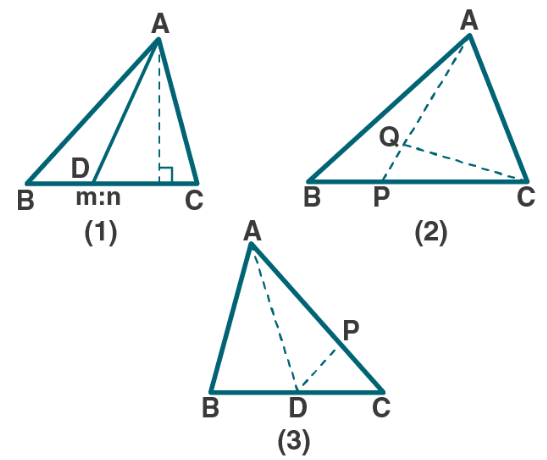Solution:

(a) Given:

From fig (1)

In ∆ABC, the point D divides the side BC in the ratio m: n.

BD: DC = m: n

To prove:

area of ∆ ABD: area of ∆ ADC = m: n

Proof:

area of ∆ ABD = ½ × base × height

ar (∆ ABD) = ½ × BD × AE ….. (1)

ar (∆ ACD) = ½ × DC × AE ….. (2)

let us divide (1) by (2)

[ar (∆ ABD) = ½ × BD × AE] / [ar (∆ ACD) = ½ × DC × AE] [ar (∆ ABD)] / [ar (∆ ACD)] = BD/DC

= m/n [it is given that, BD: DC = m: n]

Hence proved.

(b) Given:

From fig (2)

In ∆ABC, P is a point on the side BC such that PC = 2BP, and Q is a point on AP such that QA = 5 PQ.

To Find:

area of ∆AQC: area of ∆ABC

Now,

It is given that: PC = 2BP

PC/2 = BP

We know that, BC = BP + PC

Now substitute the values, we get

BC = BP + PC

= PC/2 + PC

= (PC + 2PC)/2

= 3PC/2

2BC/3 = PC

ar (∆APC) = 2/3 ar (∆ABC) …… (1)

It is given that, QA = 5PQ

QA/5 = PQ

We know that, QA= QA + PQ

So, QA = 5/6 AP

ar (∆AQC) = 5/6 ar (∆APC)

= 5/6 (2/3 ar (∆ABC)) [From (1)]

ar (∆AQC) = 5/9 ar (∆ABC)

ar (∆AQC)/ ar (∆AQC) = 5/9

Hence proved.

(c) Given:

From fig (3)

AD is a median of ∆ABC and P is a point in AC such that area of ∆ADP: area of ∆ABD = 2:3

To Find:

(i) AP: PC

(ii) area of ∆PDC: area of ∆ABC

Now,

(i) we know that AD is the median of ∆ABC

ar (∆ABD) = ar (∆ADC) = ½ ar (∆ABC) ……. (1)

It is given that,

ar (∆ADP): ar (∆ABD) = 2: 3

AP: AC = 2: 3

AP/AC = 2/3

AP = 2/3 AC

Now,

PC = AC – AP

= AC – 2/3 AC

= (3AC-2AC)/3

= AC/3 …….. (2)

So,

AP/PC = (2/3 AC) / (AC/3)

= 2/1

AP: PC = 2:1

(ii) we know that from (2)

PC = AC/3

PC/AC = 1/3

So,

= 1/3

ar (∆PDC)/1/2 ar (∆ABC) = 1/3

ar (∆PDC)/ar (∆ABC) = 1/3 × ½

= 1/6

ar (∆PDC): ar (∆ABC) = 1: 6

Hence proved.

10. (a) In the figure (1) given below, area of parallelogram ABCD is 29 cm2. Calculate the height of parallelogram ABEF if AB = 5.8 cm

(b) In the figure (2) given below, area of ∆ABD is 24 sq. units. If AB = 8 units, find the height of ABC.

(c) In the figure (3) given below, E and F are mid points of sides AB and CD respectively of parallelogram ABCD. If the area of parallelogram ABC is 36 cm2.

(i) State the area of ∆ APD.

(ii) Name the parallelogram whose area is equal to the area of ∆ APD.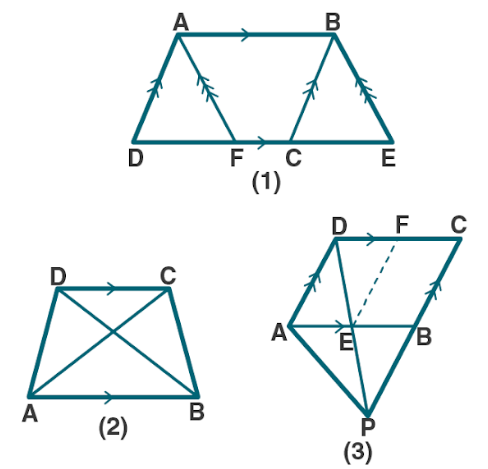Solution:

(a) Given:

From fig (1)

ar ||gm ABCD = 29cm2

To find:

Height of parallelogram ABEF if AB = 5.8 cm

Now, let us find

We know that ||gm ABCD and ||gm ABEF with equal bases and between the same parallels so that there area are same.

ar (||gm ABEF) = ar (||gm ABCD)

ar (||gm ABEF) = 29cm2 ….. (1) [Since, ar ||gm ABCD = 29cm2]

also, ar (||gm ABEF = base × height)

29 = AB × height [From (1)]

29 = 5.8 × height

Height = 29/5.8

= 5

∴ Height of parallelogram ABEF is 5cm

(b) Given:

From fig (2)

area of ∆ABD is 24 sq. units. AB = 8 units

To find:

Height of ABC

Now, let us find

We know that ar ∆ABD = 24 sq. units …… (1)

So, ar ∆ABD = ∆ABC ….. (2)

From (1) and (2)

ar ∆ABC = 24 sq. units

½ × AB × height = 24

½ × 8 × height = 24

4 × height = 24

Height = 24/4

= 6

∴ Height of ∆ABC = 6 sq. units

(c) Given:

From fig (3)

In ||gm ABCD, E and F are mid points of sides AB and CD respectively.

ar (||gm ABCD) = 36cm2

To find:

(i) State the area of ∆ APD.

(ii) Name the parallelogram whose area is equal to the area of ∆ APD.

Now, let us find

(i) we know that ∆ APD and ||gm ABCD are on the same base AD and between the same parallel lines AD and BC.

ar (∆ APD) = ½ ar (||gm ABCD) ….. (1)

ar (||gm ABCD) = 36cm2 ….. (2)

From (1) and (2)

ar (∆ APD) = ½ × 36

= 18cm2

(ii) we know that E and F are mid-points of AB and CD

In ∆CPD, EF || PC

Also, EF bisects the ||gm ABCD in two eual parts.

So, EF || AD and AE || DF

AEFD is a parallelogram.

ar (||gm AEFD) = ½ ar (||gm ABCD) ……. (3)

From (1) and (3)

ar (∆APD) = ar (||gm AEFD)

∴ AEFD is the required parallelogram which is equal to area of ∆APD.

11. (a) In the figure (1) given below, ABCD is a parallelogram. Points P and Q on BC trisect BC into three equal parts. Prove that :

area of ∆APQ = area of ∆DPQ = 1/6 (area of ||gm ABCD)

(b) In the figure (2) given below, DE is drawn parallel to the diagonal AC of the quadrilateral ABCD to meet BC produced at the point E. Prove that area of quad. ABCD = area of ∆ABE.

(c) In the figure (3) given below, ABCD is a parallelogram. O is any point on the diagonal AC of the parallelogram. Show that the area of ∆AOB is equal to the area of ∆AOD.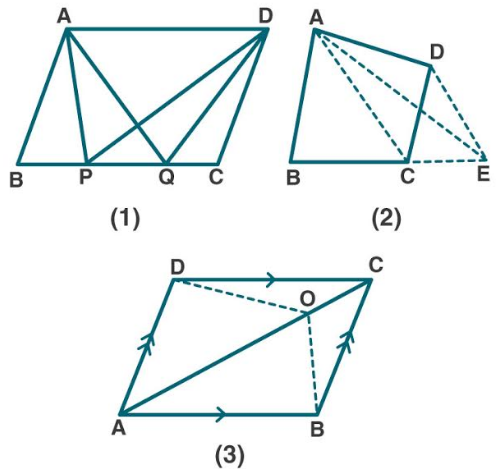Solution:

(a) Given:

From fig (1)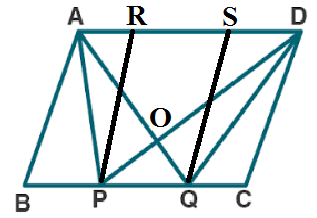In ||gm ABCD, points P and Q trisect BC into three equal parts.

To prove:

area of ∆APQ = area of ∆DPQ = 1/6 (area of ||gm ABCD)

Firstly, let us construct: through P and Q, draw PR and QS parallel to AB and CD.

Proof:

ar (∆APD) = ar (∆AQD) [Since, ∆APD and ∆AQD lie on the same base AD and between the same parallel lines AD and BC]

ar (∆APD) – ar (∆AOD) = ar (∆AQD) – ar (∆AOD) [On subtracting ar ∆AOD on both sides]

ar (∆APO) = ar (∆OQD) ….. (1)

ar (∆APO) + ar (∆OPQ) = ar (∆OQD) + ar (∆OPQ) [On adding ar ∆OPQ on both sides]

ar (∆APQ) = ar (∆DPQ) …… (2)

We know that, ∆APQ and ||gm PQSR are on the same base PQ and between same parallel lines PQ and AD.

ar (∆APQ) = ½ ar (||gm PQRS) …… (3)

Now,

[ar (||gm ABCD)/ar (||gm PQRS)] = [(BC×height)/(PQ×height)] = [(3PQ×height)/(1PQ×hight)]

ar (||gm PQRS) = 1/3 ar (||gm ABCD) …. (4)

by using (2), (3), (4), we get

ar (∆APQ) = ar (∆DPQ)

= ½ ar (||gm PQRS)

= ½ × 1/3 ar (||gm ABCD)

= 1/6 ar (||gm ABCD)

Hence proved.

(b) Given:

In the figure (2) given below, DE || AC the diagonal of the quadrilateral ABCD to meet at point E on producing BC. Join AC, AE.

To prove:

area of quad. ABCD = area of ∆ABE

Proof:

We know that, ∆ACE and ∆ADE are on the same base AC and between the same parallelogram.

Now by adding ar (∆ABC) on both sides, we get

ar (∆ACE) + ar (∆ABC) = ar (∆ADC) + ar (∆ABC)

ar (∆ ABE) = ar quad. ABCD

Hence proved.

(c) Given:

From fig (3)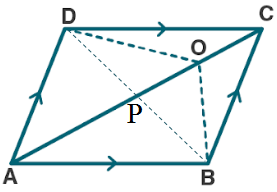In ||gm ABCD, O is any point on diagonal AC.

To prove:

area of ∆AOB is equal to the area of ∆AOD

Proof:

Let us join BD which meets AC at P.

In ∆ABD, AP is the median.

ar (∆ABP) = ar (∆ADP) ….. (1)

similarly, ar (∆PBO) = ar (∆PDO) …. (2)

now add (1) and (2), we get

ar (∆ABO) = ar (∆ADO) …. (3)

so,

∆AOB = ar ∆AOD

Hence proved.

12. (a) In the figure given, ABCD and AEFG are two parallelograms.

Prove that area of || gm ABCD = area of || gm AEFG.

(b) In the fig. (2) Given below, the side AB of the parallelogram ABCD is produced to E. A straight line through A is drawn parallel to CE to meet CB produced at F and parallelogram BFGE is Completed prove that area of || gm BFGE=Area of || gm ABCD.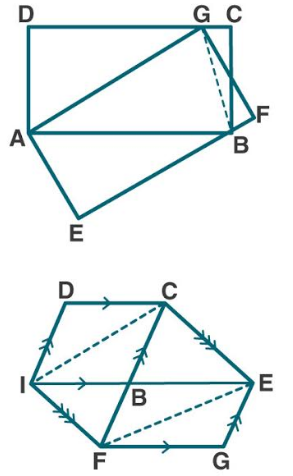(c) In the figure (3) given below AB || DC || EF, AD || BE and DE || AF. Prove the area of DEFH is equal to the area of ABCD.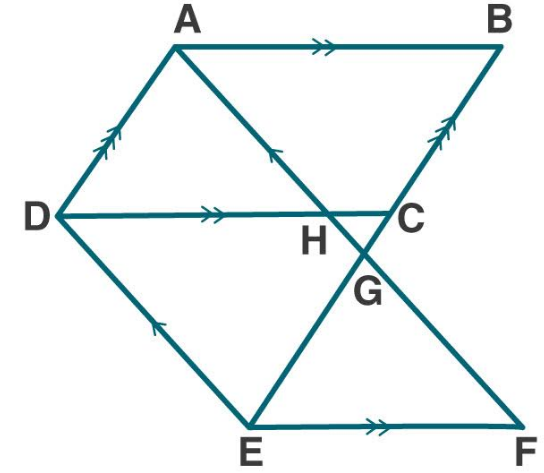Solution:

(a) Given:

From fig (1)

ABCD and AEFG are two parallelograms as shown in the figure.

To prove:

area of || gm ABCD = area of || gm AEFG

Proof:

let us join BG.

We know that,

ar (∆ABG) = ½ (ar ||gm ABCD) …… (1)

Similarly,

ar (∆ABG) = ½ (ar ||gm AEFG) …. (2)

From (1) and (2)

½ (ar ||gm ABCD) = ½ (ar ||gm AEFG)

So,

ar ||gm ABCD = ar ||gm AEFG)

Hence proved.

(b) Given:

From fig (2)

A parallelogram ABCD in which AB is produced to E. A straight line through A is drawn parallel to CE to meet CB produced at F and parallelogram BFGE is Completed.

To prove:

area of || gm BFGE=Area of || gm ABCD

Proof:

Let us join AC and EF.

We know that,

ar (∆AFC) = ar (∆AFE) ….. (1)

now subtract ar (∆ABF) on both sides, we get

ar (∆AFC) – ar (∆ABF) = ar (∆AFE) – ar (∆ABF)

Or ar (∆ABC) = ar (∆BEF)

Now multiply by 2 on both sides, we get

2. ar (∆ABC) = 2. ar (∆BEF)

Or ar (||gm ABCD) = ar (||gm BFGE)

Hence proved.

(c) Given:

From fig (3)

AB || DC || EF, AD || BE and DE || AF

To prove:

area of DEFH = area of ABCD

Proof:

We know that,

DE || AF and AD || BE

It is given that ADEG is a parallelogram.

So,

ar (||gm ABCD) = ar (||gm ADEG) ….. (1)

Again, DEFG is a parallelogram.

ar (||gm DEFH) = ar (||gm ADEG) ….. (2)

From (1) and (2)

ar (||gm ABCD) = ar (||gm DEFH)

Or ar ABCD = ar DEFH

Hence proved.

13. Any point D is taken on the side BC of, a ∆ ABC and AD is produced to E such that AD=DE, prove that area of ∆ BCE = area of ∆ ABC.
Solution:

Given:

In ∆ABC, D is taken on the side BC.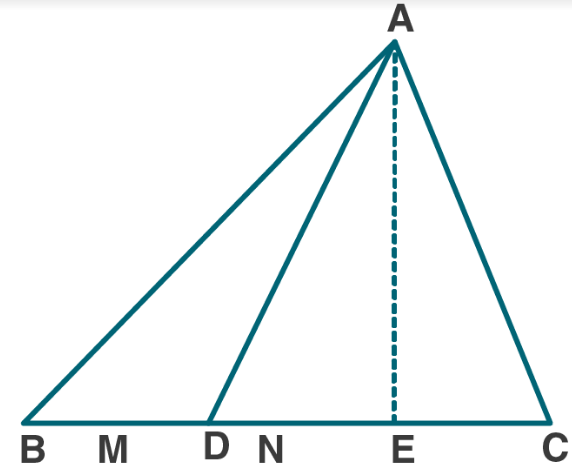To prove:

area of ∆ BCE = area of ∆ ABC

Proof:

In ∆ABE, it is given that AD = DE

So, BD is the median of ∆ABE

ar (∆ABD) = ar (∆BED) ….. (1)

similarly,

In ∆ACE, CD is the median of ∆ACE

ar (∆ACD) = ar (∆CED) ….. (2)

By adding (1) and (2), we get

ar (∆ABD) + ar (∆ACD) = ar (∆BED) + ar (∆CED)

Or ar (∆ABC) = ar (∆BCE)

Hence proved.

14. ABCD is a rectangle and P is mid-point of AB. DP is produced to meet CB at Q. Prove that area of rectangle ∆BCD = area of ∆ DQC.
Solution:

Given:

ABCD is a rectangle and P is mid-point of AB. DP is produced to meet CB at Q.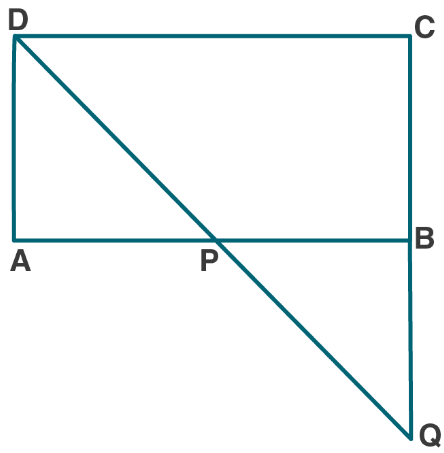To prove:

area of rectangle ∆BCD = area of ∆ DQC

Proof:

In ∆APD and ∆BQP

AP = BP [Since, D is the mid-point of AB]

∠DAP = ∠QBP [each angle is 90o]

∠APD = ∠BPQ [vertically opposite angles]

So, ∆APD ≅ ∆BQP [By using ASA postulate]

ar (∆APD) = ar (∆BQP)

Now,

ar ABCD = ar (∆APD) + ar PBCD

= ar (∆BQP) + ar PBCD

= ar (∆DQC)

Hence proved.

15. (a) In the figure (1) given below, the perimeter of parallelogram is 42 cm. Calculate the lengths of the sides of the parallelogram.

(b) In the figure (2) given below, the perimeter of ∆ ABC is 37 cm. If the lengths of the altitudes AM, BN and CL are 5x, 6x, and 4x respectively, Calculate the lengths of the sides of ∆ABC.

(c) In the fig. (3) Given below, ABCD is a parallelogram. P is a point on DC such that area of ∆DAP = 25 cm² and area of ∆BCP = 15 cm². Find

(i) area of || gm ABCD

(ii) DP: PC.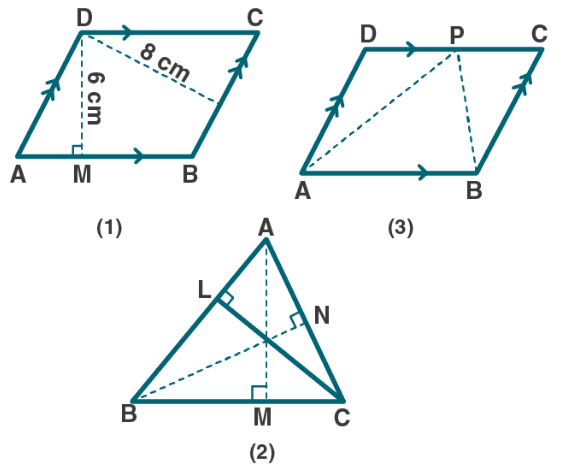Solution:

(a) Given:

The perimeter of parallelogram ABCD = 42 cm

To find:

Lengths of the sides of the parallelogram ABCD.

From fig (1)

We know that,

AB = P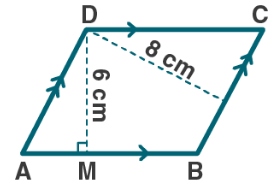Then, perimeter of ||gm ABCD = 2 (AB + BC)

42 = 2(P + BC)

42/2 = P + BC

21 = P + BC

BC = 21 – P

So, ar (||gm ABCD) = AB × DM

= P × 6

= 6P ………. (1)

Again, ar (||gm ABCD) = BC × DN

= (21 – P) × 8

= 8(21 – P) ………. (2)

From (1) and (2), we get

6P = 8(21 – P)

6P = 168 – 8P

6P + 8P = 168

14P = 168

P = 168/14

= 12

Hence, sides of ||gm are

AB = 12cm and BC = (21 – 12)cm = 9cm

(b) Given:

The perimeter of ∆ ABC is 37 cm. The lengths of the altitudes AM, BN and CL are 5x, 6x, and 4x respectively.

To find:

Lengths of the sides of ∆ABC. i.e., BC, CA and AB.

Let us consider BC = P and CA = Q

From fig (2),

Then, perimeter of ∆ABC = AB + BC + CA

37 = AB + P + Q

AB = 37 – P – Q

Area (∆ABC) = ½ × base × height

= ½ × BC × AM = ½ × CA × BN = ½ × AB × CL

= ½ × P × 5x = ½ × Q × 6x = ½ (37 – P – Q) × 4x

= 5P/2 = 3Q = 2(37 – P – Q)

Let us consider first two parts:

5P/2 = 3Q

5P = 6Q

5P – 6Q = 0 …… (1)

25P – 30Q (multiplying by 5)….. (2)

Let us consider second and third parts:

3Q = 2(37 – P – Q)

3Q = 74 – 2P – 2Q

3Q + 2Q + 2P = 74

2P + 5Q = 74 ……. (3)

12P + 30Q = 444 (multiplying by 6)……. (4)

By adding (2) and (4), we get

37P = 444

P = 444/37

= 12

Now, substitute the value of P in equation (1), we get

5P – 6Q = 0

5(12) – 6Q = 0

60 = 6Q

Q = 60/6

= 10

Hence, BC = P = 12cm

CA = Q = 10cm

And AB = 37 – P – Q = 37 – 12 – 10 = 15cm

(c) Given:

ABCD is a parallelogram. P is a point on DC such that area of ∆DAP = 25 cm² and area of ∆BCP = 15 cm².

To Find:

(i) area of || gm ABCD

(ii) DP: PC

Now let us find,

From fig (3)

(i) we know that,

ar (∆APB) = ½ ar (||gm ABCD)

Then,

½ ar (||gm ABCD) = ar (∆DAP) + ar (∆BCP)

= 25 + 15

= 40cm2

So, ar (||gm ABCD) = 2 × 40 = 80cm2

(ii) we know that,

∆ADP and ∆BCP are on the same base CD and between same parallel lines CD and AB.

ar (∆DAP)/ar(∆BCP) = DP/PC

25/15 = DP/PC

5/3 = DP/PC

So, DP: PC = 5: 3

16. In the adjoining figure, E is mid-point of the side AB of a triangle ABC and EBCF is a parallelogram. If the area of ∆ ABC is 25 sq. units, find the area of || gm EBCF.

Solution: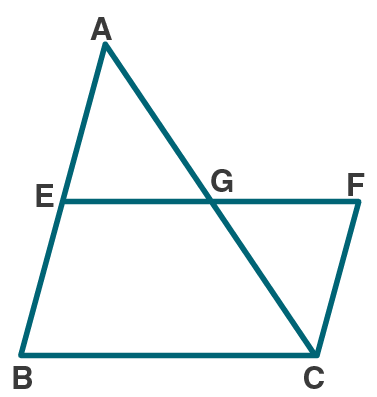Let us consider EF, side of ||gm BCFE meets AC at G.

We know that, E is the mid-point and EF || BC

G is the mid-point of AC.

So,

AG = GC

Now, in ∆AEG and ∆CFG,

The alternate angles are: ∠EAG, ∠GCF

Vertically opposite angles are: ∠EGA = ∠CGF

So, AG = GC

Proved.

∴ ∆AEG ≅ ∆CFG

ar (∆AEG) = ar (∆CFG)

Now,

ar (||gm EBCF) = ar BCGE + ar (∆CFG)

= ar BCGE + ar (∆AEG)

= ar (∆ABC)

We know that, ar (∆ABC) = 25sq. units

Hence, ar (||gm EBCF) = 25sq. units

17. (a) In the figure (1) given below, BC || AE and CD || BE. Prove that: area of ∆ABC= area of ∆EBD.

(b) In the figure (2) given below, ABC is right angled triangle at A. AGFB is a square on the side AB and BCDE is a square on the hypotenuse BC. If AN ⊥ ED, prove that:

(i) ∆BCF ≅ ∆ ABE.

(ii) area of square ABFG = area of rectangle BENM.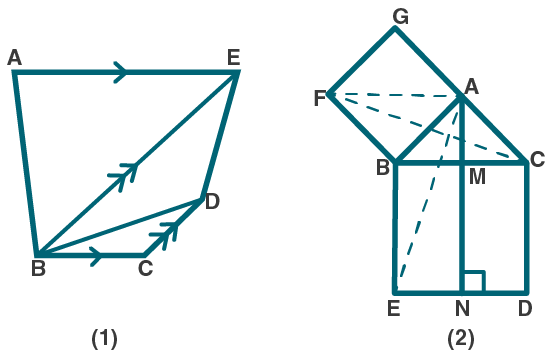Solution:

(a) Given:

From fig (1)

BC || AE and CD || BE

To prove:

area of ∆ABC= area of ∆EBD

Proof: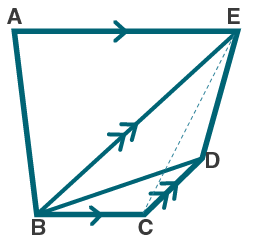By joining CE.

We know that, from ∆ABC and ∆EBC

ar (∆ABC) = ar (∆EBC) ….. (1)

From EBC and ∆EBD

ar (∆EBC) = ar (∆EBD) …… (2)

From (1) and (2), we get

ar (∆ABC) = ar (∆EBD)

Hence proved.

(b) Given:

ABC is right angled triangle at A. Squares AGFB and BCDE are drawn on the side AB and hypotenuse BC of ∆ABC. AN ⊥ ED which meets BC at M.

To prove:

(i) ∆BCF ≅ ∆ ABE.

(ii) area of square ABFG = area of rectangle BENM

From the figure (2)

(i) ∠FBC = ∠FBA + ∠ABC

So,

∠FBC = 90o + ∠ABC ….. (1)

∠ABE = ∠EAC + ∠ABC

So,

∠ABE = 90o + ∠ABC ….. (2)

From (1) and (2), we get

∠FBC = ∠ABE ….. (3)

So, BC = BE

Now, in ∆BCF and ∆ABE

BF = AB

By using SAS axiom rule of congruency,

∴ ∆BCF ≅ ∆ ABE

Hence proved.

(ii) we know that,

∆BCF ≅ ∆ ABE

So, ar (∆BCF) = ar (∆ABE) ….. (4)

∠BAG + ∠BAC = 90o + 90o

= 180o

So, GAC is a straight line.

Now, from ∆BCF and square AGFB

ar (∆BCF) = ½ ar (square AGFB) …. (5)

From ∆ABE and rectangle BENM

ar (∆ABE) = ½ ar (rectangle BENM) ….. (6)

From (4), (5) and (6)

½ ar (square AGFB) = ½ ar (rectangle BENM)

ar (square AGFB) = ar (rectangle BENM)

Hence proved.

EXERCISE 14

1. Prove that the line segment joining the mid-points of a pair of opposite sides of a parallelogram divides it into two equal parallelograms.

Solution:

Let us consider ABCD be a parallelogram in which E and F are mid-points of AB and CD. Join EF.

To prove: ar (|| AEFD) = ar (|| EBCF)Let us construct DG ⊥ AG and let DG = h where, h is the altitude on side AB.

Proof:

ar (|| ABCD) = AB × h

ar (|| AEFD) = AE × h

= ½ AB × h ….. (1) [Since, E is the mid-point of AB]

ar (|| EBCF) = EF × h

= ½ AB × h …… (2) [Since, E is the mid-point of AB]

From (1) and (2)

ar (|| ABFD) = ar (|| EBCF)

Hence proved.

2. Prove that the diagonals of a parallelogram divide it into four triangles of equal area.

Solution:

Let us consider in a parallelogram ABCD the diagonals AC and BD are cut at point O.

To prove: ar (∆AOB) = ar (∆BOC) = ar (∆COD) = ar (∆AOD)Proof:

In parallelogram ABCD the diagonals bisect each other.

AO = OC

In ∆ACD, O is the mid-point of AC. DO is the median.

ar (∆AOD) = ar (COD) ….. (1) [Median of ∆ divides it into two triangles of equal arreas]

Similarly, in ∆ ABC

ar (∆AOB) = ar (∆COB) ….. (2)

ar (∆AOD) = ar (∆AOB) …. (3)

In ∆CDB

ar (∆COD) = ar (∆COB) …. (4)

From (1), (2), (3) and (4)

ar (∆AOB) = ar (∆BOC) = ar (∆COD) = ar (∆AOD)

Hence proved.

3. (a) In the figure (1) given below, AD is median of ∆ABC and P is any point on AD. Prove that

(i) Area of ∆PBD = area of ∆PDC.

(ii) Area of ∆ABP = area of ∆ACP.

(b) In the figure (2) given below, DE || BC. Prove that

(i) area of ∆ACD = area of ∆ ABE.

(ii) Area of ∆OBD = area of ∆OCE.Solution:

(a) Given:

∆ABC in which AD is the median. P is any point on AD. Join PB and PC.

To prove:

(i) Area of ∆PBD = area of ∆PDC.

(ii) Area of ∆ABP = area of ∆ACP.

Proof:

From fig (1)

AD is a median of ∆ABC

So, ar (∆ABD) = ar (∆ADC) …. (1)

Also, PD is the median of ∆BPD

Similarly, ar (∆PBD) = ar (∆PDC) …. (2)

Now, let us subtract (2) from (1), we get

ar (∆ABD) – ar (∆PBD) = ar (∆ADC) – ar (∆PDC)

Or ar (∆ABP) = ar (∆ACP)

Hence proved.

(b) Given:

∆ABC in which DE || BC

To prove:

(i) area of ∆ACD = area of ∆ ABE.

(ii) Area of ∆OBD = area of ∆OCE.

Proof:

From fig (2)

∆DEC and ∆BDE are on the same base DE and between the same || line DE and BE.

ar (∆DEC) = ar (∆BDE)

ar (∆ACD) = ar (∆ABE)

Hence proved.

Similarly, ar (∆DEC) = ar (∆BDE)

Subtract ar (∆DOE) from both sides, we get

ar (∆DEC) – ar (∆DOE) = ar (∆BDE) – ar (∆DOE)

ar (∆OBD) = ar (∆OCE)

Hence proved.

4. (a) In the figure (1) given below, ABCD is a parallelogram and P is any point in BC. Prove that: Area of ∆ABP + area of ∆DPC = Area of ∆APD.

(b) In the figure (2) given below, O is any point inside a parallelogram ABCD. Prove that:

(i) area of ∆OAB + area of ∆OCD = ½ area of || gm ABCD

(ii) area of ∆ OBC + area of ∆ OAD = ½ area of || gm ABCDSolution:

(a) Given:

From fig (1)

ABCD is a parallelogram and P is any point in BC.

To prove:

Area of ∆ABP + area of ∆DPC = Area of ∆APD

Proof:

∆APD and || gm ABCD are on the same base AD and between the same || lines AD and BC,

ar (∆APD) = ½ ar (|| gm ABCD) …. (1)

In parallelogram ABCD

ar(|| gm ABCD) = ar (∆ ABP) + ar (∆APD) + ar (∆DPC)

Now, divide both sides by 2, we get

½ ar(|| gm ABCD) = ½ ar (∆ ABP) + ½ ar (∆APD) + ½ ar (∆DPC) …. (2)

From (1) and (2)

ar (∆APD) = ½ ar (|| gm ABCD)

Substituting (2) in (1)

ar (∆APD) = ½ ar (∆ ABP) + ½ ar (∆APD) + ½ ar (∆DPC)

ar (∆APD) – ½ ar (∆APD) = ½ ar (∆ ABP) + ½ ar (∆DPC)

½ ar (∆APD) = ½ [ar (∆ ABP) + ar (∆DPC)]

ar (∆APD) = ar (∆ ABP) + ar (∆DPC)

Or ar (∆ ABP) + ar (∆DPC) = ar (∆APD)

Hence proved.

(b) Given:

From fig (2)

|| gm ABCD in which O is any point inside it.

To prove:

(i) area of ∆OAB + area of ∆OCD = ½ area of || gm ABCD

(ii) area of ∆ OBC + area of ∆ OAD = ½ area of || gm ABCD

Draw POQ || AB through O. It meets AD at P and BC at Q.

Proof:

(i) AB || PQ and AP || BQ

ABQP is a || gm

Similarly, PQCD is a || gm

Now, ∆OAB and || gm ABQP are on same base AB and between same || lines AB and PQ

ar (∆OAB) = ½ ar (||gm ABQP) …. (1)

Similarly, ar (∆OCD) = ½ ar (||gm PQCD) …. (2)

Now by adding (1) and (2)

ar (∆OAB) + ar (∆OCD) = ½ ar (|| gm ABQP) + ½ ar (|| gm PQCD)

= ½ [ar (|| gm ABQP) + ar (|| gm PQCD)]

= ½ ar (|| gm ABCD)

ar (∆OAB) + ar (∆OCD) = ½ ar (|| gm ABCD)

Hence proved.

(ii) we know that,

ar (∆OAB) + ar (∆ OBC) + ar (∆OCD) + ar (∆OAD) = ar (|| gm ABCD)

[ar (∆OAB) + ar (∆OCD)] + [ar (∆ OBC) + ar (∆OAD)] = ar (|| gm ABCD)

½ ar (|| gm ABCD) + ar (∆OBC) + ar (∆OAD) = ar (|| gm ABCD)

ar (∆OBC) + ar (∆OAD) = ar (|| gm ABCD) – ½ ar (|| gm ABCD)

ar (∆OBC) + ar (∆OAD) = ½ ar (|| gm ABCD)

Hence proved.

5. If E, F, G and H are mid-points of the sides AB, BC, CD and DA respectively of a parallelogram ABCD, prove that area of quad. EFGH = 1/2 area of || gm ABCD.

Solution:

Given:

In parallelogram ABCD, E, F, G, H are the mid-points of its sides AB, BC, CD and DA.

Join EF, FG, GH and HE.

To prove:

area of quad. EFGH = ½ area of || gm ABCDProof:

Let us join EG.

We know that, E and G are mid-points of AB and CD.

AEGD and EBCG are parallelogram

Now, || gm AEGD and ∆EHG are on the same base and between the parallel lines.

ar ∆EHG = ½ ar || gm AEGD …. (1)

Similarly,

ar ∆EFG = ½ ar || gm EBCG …. (2)

Now by adding (1) and (2)

ar ∆EHG + ar ∆EFG = ½ ar || gm AEGD + ½ ar || gm EBCG

area quad. EFGH = ½ ar || gm ABCD

Hence proved.

6. (a) In the figure (1) given below, ABCD is a parallelogram. P, Q are any two points on the sides AB and BC respectively. Prove that, area of ∆ CPD = area of ∆ AQD.(b) In the figure (2) given below, PQRS and ABRS are parallelograms and X is any point on the side BR. Show that area of ∆ AXS = ½ area of ||gm PQRS.

Solution:

(a) Given:

From fig (1)

||gm ABCD in which P is a point on AB and Q is a point on BC.

To prove:

area of ∆ CPD = area of ∆ AQD.

Proof:

∆ CPD and ||gm ABCD are on the same base CD and between the same parallels AB and CD.

ar (∆ CPD) = ½ ar (||gm ABCD) …. (1)

∆ AQD and ||gm ABCD are on the same base AD and between the same parallels AD and BC.

ar (∆AQD) = ½ ar (||gm ABCD) …. (2)

from (1) and (2)

ar (∆ CPD) = ar (∆AQD)

Hence proved.

(b) From fig (2)

Given:

PQRS and ABRS are parallelograms on the same base SR. X is any point on the side BR.

Join AX and SX.

To prove:

area of ∆ AXS = ½ area of ||gm PQRS

we know that, || gm PQRS and ABRS are on the same base SR and between the same parallels.

So, ar ||gm PQRS = ar ||gm ABRS …. (1)

we know that, ∆ AXS and || gm ABRS are on the same base AS and between the same parallels.

So, ar ∆ AXS = ½ ar ||gm ABRS

= ½ ar ||gm PQRS [From (1)]

Hence proved.

7. D, E and F are mid-point of the sides BC, CA and AB respectively of a ∆ ABC. Prove that

(i) FDCE is a parallelogram

(ii) area of ∆ DEF = ¼ area of ∆ ABC

(iii) area of || gm FDCE = ½ area of ∆ ABC

Solution:

Given:

D, E and F are mid-point of the sides BC, CA and AB respectively of a ∆ ABC.To prove:

(i) FDCE is a parallelogram

(ii) area of ∆ DEF = ¼ area of ∆ ABC

(iii) area of || gm FDCE = ½ area of ∆ ABC

Proof:

(i) F and E are mid-points of AB and AC.

So, FE || BC and FE = ½ BC ….. (1)

Also, D is mid-point of BC

CD = ½ BC …… (2)

From (1) and (2)

FE || BC and FE = CD

FE || CD and FE = CD …… (3)

Similarly,

D and F are mid-points of BC and AB.

So, DF || EC is a parallelogram.

Hence proved.

(ii) we know that, FDCE is a parallelogram.

And DE is a diagonal of ||gm FDCE

So, ar (∆ DEF) = ar (∆DEC) ….. (4)

Similarly, we know BDEF and DEAF are ||gm

So, ar (∆ DEF) = ar (∆ BDF) = ar (∆ AFE) ….. (5)

From (4) and (5)

ar (∆ DEF) = ar (∆DEC) = ar (∆ BDF) = ar (∆ AFE)

Now, ar (∆ ABC) = ar (∆ DEF) + ar (∆ DEF) + ar (∆ DEF) + ar (∆ DEF)

= 4 ar (∆ DEF)

ar (∆ DEF) = ¼ ar (∆ ABC) ….. (6)

Hence proved.

(iii) ar of || gm FDCE = ar (∆ DEF) + ar (∆ DEC)

= ar (∆ DEF) + ar (∆ DEF)

= 2 ar (∆ DEF) [From (4)]

= 2 [¼ ar (∆ ABC)] [From (6)]

ar of || gm FDCE = ½ ar of ∆ ABC

Hence proved.

8. In the given figure, D, E and F are mid points of the sides BC, CA and AB respectively of ∆ ABC. Prove that BCEF is a trapezium and area of trap. BCEF = ¾ area of ∆ ABC.Solution:

Given:

In ABC, D, E and F are mid points of the sides BC, CA and AB.

To prove:

area of trap. BCEF = ¾ area of ∆ ABC

Proof:

We know that D and E are the mid-points of BC and CA.

So, DE || AB and ½ AB

Similarly,

EF || BC and ½ BC

And FD || AC and ½ AC

∴ BDEF, CDFE, AFDE are parallelograms which are equal in area.

ED, DF, EF are diagonals of these ||gm which divides the corresponding parallelogram into two triangles equal in area.

Hence, BCEF is a trapezium.

area of trap. BCEF = ¾ area of ∆ ABC

9. (a) In the figure (1) given below, the point D divides the side BC of ∆ABC in the ratio m: n. Prove that area of ∆ ABD: area of ∆ ADC = m: n.

(b) In the figure (2) given below, P is a point on the side BC of ∆ABC such that PC = 2BP, and Q is a point on AP such that QA = 5 PQ, find area of ∆AQC: area of ∆ABC.

(c) In the figure (3) given below, AD is a median of ∆ABC and P is a point in AC such that area of ∆ADP: area of ∆ABD = 2:3. Find

(i) AP: PC

(ii) area of ∆PDC: area of ∆ABC.Solution:

(a) Given:

From fig (1)

In ∆ABC, the point D divides the side BC in the ratio m: n.

BD: DC = m: n

To prove:

area of ∆ ABD: area of ∆ ADC = m: n

Proof:

area of ∆ ABD = ½ × base × height

ar (∆ ABD) = ½ × BD × AE ….. (1)

ar (∆ ACD) = ½ × DC × AE ….. (2)

let us divide (1) by (2)

[ar (∆ ABD) = ½ × BD × AE] / [ar (∆ ACD) = ½ × DC × AE] [ar (∆ ABD)] / [ar (∆ ACD)] = BD/DC

= m/n [it is given that, BD: DC = m: n]

Hence proved.

(b) Given:

From fig (2)

In ∆ABC, P is a point on the side BC such that PC = 2BP, and Q is a point on AP such that QA = 5 PQ.

To Find:

area of ∆AQC: area of ∆ABC

Now,

It is given that: PC = 2BP

PC/2 = BP

We know that, BC = BP + PC

Now substitute the values, we get

BC = BP + PC

= PC/2 + PC

= (PC + 2PC)/2

= 3PC/2

2BC/3 = PC

ar (∆APC) = 2/3 ar (∆ABC) …… (1)

It is given that, QA = 5PQ

QA/5 = PQ

We know that, QA= QA + PQ

So, QA = 5/6 AP

ar (∆AQC) = 5/6 ar (∆APC)

= 5/6 (2/3 ar (∆ABC)) [From (1)]

ar (∆AQC) = 5/9 ar (∆ABC)

ar (∆AQC)/ ar (∆AQC) = 5/9

Hence proved.

(c) Given:

From fig (3)

AD is a median of ∆ABC and P is a point in AC such that area of ∆ADP: area of ∆ABD = 2:3

To Find:

(i) AP: PC

(ii) area of ∆PDC: area of ∆ABC

Now,

(i) we know that AD is the median of ∆ABC

ar (∆ABD) = ar (∆ADC) = ½ ar (∆ABC) ……. (1)

It is given that,

ar (∆ADP): ar (∆ABD) = 2: 3

AP: AC = 2: 3

AP/AC = 2/3

AP = 2/3 AC

Now,

PC = AC – AP

= AC – 2/3 AC

= (3AC-2AC)/3

= AC/3 …….. (2)

So,

AP/PC = (2/3 AC) / (AC/3)

= 2/1

AP: PC = 2:1

(ii) we know that from (2)

PC = AC/3

PC/AC = 1/3

So,

= 1/3

ar (∆PDC)/1/2 ar (∆ABC) = 1/3

ar (∆PDC)/ar (∆ABC) = 1/3 × ½

= 1/6

ar (∆PDC): ar (∆ABC) = 1: 6

Hence proved.

10. (a) In the figure (1) given below, area of parallelogram ABCD is 29 cm2. Calculate the height of parallelogram ABEF if AB = 5.8 cm

(b) In the figure (2) given below, area of ∆ABD is 24 sq. units. If AB = 8 units, find the height of ABC.

(c) In the figure (3) given below, E and F are mid points of sides AB and CD respectively of parallelogram ABCD. If the area of parallelogram ABC is 36 cm2.

(i) State the area of ∆ APD.

(ii) Name the parallelogram whose area is equal to the area of ∆ APD.Solution:

(a) Given:

From fig (1)

ar ||gm ABCD = 29cm2

To find:

Height of parallelogram ABEF if AB = 5.8 cm

Now, let us find

We know that ||gm ABCD and ||gm ABEF with equal bases and between the same parallels so that there area are same.

ar (||gm ABEF) = ar (||gm ABCD)

ar (||gm ABEF) = 29cm2 ….. (1) [Since, ar ||gm ABCD = 29cm2]

also, ar (||gm ABEF = base × height)

29 = AB × height [From (1)]

29 = 5.8 × height

Height = 29/5.8

= 5

∴ Height of parallelogram ABEF is 5cm

(b) Given:

From fig (2)

area of ∆ABD is 24 sq. units. AB = 8 units

To find:

Height of ABC

Now, let us find

We know that ar ∆ABD = 24 sq. units …… (1)

So, ar ∆ABD = ∆ABC ….. (2)

From (1) and (2)

ar ∆ABC = 24 sq. units

½ × AB × height = 24

½ × 8 × height = 24

4 × height = 24

Height = 24/4

= 6

∴ Height of ∆ABC = 6 sq. units

(c) Given:

From fig (3)

In ||gm ABCD, E and F are mid points of sides AB and CD respectively.

ar (||gm ABCD) = 36cm2

To find:

(i) State the area of ∆ APD.

(ii) Name the parallelogram whose area is equal to the area of ∆ APD.

Now, let us find

(i) we know that ∆ APD and ||gm ABCD are on the same base AD and between the same parallel lines AD and BC.

ar (∆ APD) = ½ ar (||gm ABCD) ….. (1)

ar (||gm ABCD) = 36cm2 ….. (2)

From (1) and (2)

ar (∆ APD) = ½ × 36

= 18cm2

(ii) we know that E and F are mid-points of AB and CD

In ∆CPD, EF || PC

Also, EF bisects the ||gm ABCD in two eual parts.

So, EF || AD and AE || DF

AEFD is a parallelogram.

ar (||gm AEFD) = ½ ar (||gm ABCD) ……. (3)

From (1) and (3)

ar (∆APD) = ar (||gm AEFD)

∴ AEFD is the required parallelogram which is equal to area of ∆APD.

11. (a) In the figure (1) given below, ABCD is a parallelogram. Points P and Q on BC trisect BC into three equal parts. Prove that :

area of ∆APQ = area of ∆DPQ = 1/6 (area of ||gm ABCD)

(b) In the figure (2) given below, DE is drawn parallel to the diagonal AC of the quadrilateral ABCD to meet BC produced at the point E. Prove that area of quad. ABCD = area of ∆ABE.

(c) In the figure (3) given below, ABCD is a parallelogram. O is any point on the diagonal AC of the parallelogram. Show that the area of ∆AOB is equal to the area of ∆AOD.Solution:

(a) Given:

From fig (1)In ||gm ABCD, points P and Q trisect BC into three equal parts.

To prove:

area of ∆APQ = area of ∆DPQ = 1/6 (area of ||gm ABCD)

Firstly, let us construct: through P and Q, draw PR and QS parallel to AB and CD.

Proof:

ar (∆APD) = ar (∆AQD) [Since, ∆APD and ∆AQD lie on the same base AD and between the same parallel lines AD and BC]

ar (∆APD) – ar (∆AOD) = ar (∆AQD) – ar (∆AOD) [On subtracting ar ∆AOD on both sides]

ar (∆APO) = ar (∆OQD) ….. (1)

ar (∆APO) + ar (∆OPQ) = ar (∆OQD) + ar (∆OPQ) [On adding ar ∆OPQ on both sides]

ar (∆APQ) = ar (∆DPQ) …… (2)

We know that, ∆APQ and ||gm PQSR are on the same base PQ and between same parallel lines PQ and AD.

ar (∆APQ) = ½ ar (||gm PQRS) …… (3)

Now,

[ar (||gm ABCD)/ar (||gm PQRS)] = [(BC×height)/(PQ×height)] = [(3PQ×height)/(1PQ×hight)]

ar (||gm PQRS) = 1/3 ar (||gm ABCD) …. (4)

by using (2), (3), (4), we get

ar (∆APQ) = ar (∆DPQ)

= ½ ar (||gm PQRS)

= ½ × 1/3 ar (||gm ABCD)

= 1/6 ar (||gm ABCD)

Hence proved.

(b) Given:

In the figure (2) given below, DE || AC the diagonal of the quadrilateral ABCD to meet at point E on producing BC. Join AC, AE.

To prove:

area of quad. ABCD = area of ∆ABE

Proof:

We know that, ∆ACE and ∆ADE are on the same base AC and between the same parallelogram.

Now by adding ar (∆ABC) on both sides, we get

ar (∆ACE) + ar (∆ABC) = ar (∆ADC) + ar (∆ABC)

ar (∆ ABE) = ar quad. ABCD

Hence proved.

(c) Given:

From fig (3)In ||gm ABCD, O is any point on diagonal AC.

To prove:

area of ∆AOB is equal to the area of ∆AOD

Proof:

Let us join BD which meets AC at P.

In ∆ABD, AP is the median.

ar (∆ABP) = ar (∆ADP) ….. (1)

similarly, ar (∆PBO) = ar (∆PDO) …. (2)

now add (1) and (2), we get

ar (∆ABO) = ar (∆ADO) …. (3)

so,

∆AOB = ar ∆AOD

Hence proved.

12. (a) In the figure given, ABCD and AEFG are two parallelograms.

Prove that area of || gm ABCD = area of || gm AEFG.

(b) In the fig. (2) Given below, the side AB of the parallelogram ABCD is produced to E. A straight line through A is drawn parallel to CE to meet CB produced at F and parallelogram BFGE is Completed prove that area of || gm BFGE=Area of || gm ABCD.(c) In the figure (3) given below AB || DC || EF, AD || BE and DE || AF. Prove the area of DEFH is equal to the area of ABCD.Solution:

(a) Given:

From fig (1)

ABCD and AEFG are two parallelograms as shown in the figure.

To prove:

area of || gm ABCD = area of || gm AEFG

Proof:

let us join BG.

We know that,

ar (∆ABG) = ½ (ar ||gm ABCD) …… (1)

Similarly,

ar (∆ABG) = ½ (ar ||gm AEFG) …. (2)

From (1) and (2)

½ (ar ||gm ABCD) = ½ (ar ||gm AEFG)

So,

ar ||gm ABCD = ar ||gm AEFG)

Hence proved.

(b) Given:

From fig (2)

A parallelogram ABCD in which AB is produced to E. A straight line through A is drawn parallel to CE to meet CB produced at F and parallelogram BFGE is Completed.

To prove:

area of || gm BFGE=Area of || gm ABCD

Proof:

Let us join AC and EF.

We know that,

ar (∆AFC) = ar (∆AFE) ….. (1)

now subtract ar (∆ABF) on both sides, we get

ar (∆AFC) – ar (∆ABF) = ar (∆AFE) – ar (∆ABF)

Or ar (∆ABC) = ar (∆BEF)

Now multiply by 2 on both sides, we get

2. ar (∆ABC) = 2. ar (∆BEF)

Or ar (||gm ABCD) = ar (||gm BFGE)

Hence proved.

(c) Given:

From fig (3)

AB || DC || EF, AD || BE and DE || AF

To prove:

area of DEFH = area of ABCD

Proof:

We know that,

DE || AF and AD || BE

It is given that ADEG is a parallelogram.

So,

ar (||gm ABCD) = ar (||gm ADEG) ….. (1)

Again, DEFG is a parallelogram.

ar (||gm DEFH) = ar (||gm ADEG) ….. (2)

From (1) and (2)

ar (||gm ABCD) = ar (||gm DEFH)

Or ar ABCD = ar DEFH

Hence proved.

13. Any point D is taken on the side BC of, a ∆ ABC and AD is produced to E such that AD=DE, prove that area of ∆ BCE = area of ∆ ABC.
Solution:

Given:

In ∆ABC, D is taken on the side BC.To prove:

area of ∆ BCE = area of ∆ ABC

Proof:

In ∆ABE, it is given that AD = DE

So, BD is the median of ∆ABE

ar (∆ABD) = ar (∆BED) ….. (1)

similarly,

In ∆ACE, CD is the median of ∆ACE

ar (∆ACD) = ar (∆CED) ….. (2)

By adding (1) and (2), we get

ar (∆ABD) + ar (∆ACD) = ar (∆BED) + ar (∆CED)

Or ar (∆ABC) = ar (∆BCE)

Hence proved.

14. ABCD is a rectangle and P is mid-point of AB. DP is produced to meet CB at Q. Prove that area of rectangle ∆BCD = area of ∆ DQC.
Solution:

Given:

ABCD is a rectangle and P is mid-point of AB. DP is produced to meet CB at Q.To prove:

area of rectangle ∆BCD = area of ∆ DQC

Proof:

In ∆APD and ∆BQP

AP = BP [Since, D is the mid-point of AB]

∠DAP = ∠QBP [each angle is 90o]

∠APD = ∠BPQ [vertically opposite angles]

So, ∆APD ≅ ∆BQP [By using ASA postulate]

ar (∆APD) = ar (∆BQP)

Now,

ar ABCD = ar (∆APD) + ar PBCD

= ar (∆BQP) + ar PBCD

= ar (∆DQC)

Hence proved.

15. (a) In the figure (1) given below, the perimeter of parallelogram is 42 cm. Calculate the lengths of the sides of the parallelogram.

(b) In the figure (2) given below, the perimeter of ∆ ABC is 37 cm. If the lengths of the altitudes AM, BN and CL are 5x, 6x, and 4x respectively, Calculate the lengths of the sides of ∆ABC.

(c) In the fig. (3) Given below, ABCD is a parallelogram. P is a point on DC such that area of ∆DAP = 25 cm² and area of ∆BCP = 15 cm². Find

(i) area of || gm ABCD

(ii) DP: PC.Solution:

(a) Given:

The perimeter of parallelogram ABCD = 42 cm

To find:

Lengths of the sides of the parallelogram ABCD.

From fig (1)

We know that,

AB = PThen, perimeter of ||gm ABCD = 2 (AB + BC)

42 = 2(P + BC)

42/2 = P + BC

21 = P + BC

BC = 21 – P

So, ar (||gm ABCD) = AB × DM

= P × 6

= 6P ………. (1)

Again, ar (||gm ABCD) = BC × DN

= (21 – P) × 8

= 8(21 – P) ………. (2)

From (1) and (2), we get

6P = 8(21 – P)

6P = 168 – 8P

6P + 8P = 168

14P = 168

P = 168/14

= 12

Hence, sides of ||gm are

AB = 12cm and BC = (21 – 12)cm = 9cm

(b) Given:

The perimeter of ∆ ABC is 37 cm. The lengths of the altitudes AM, BN and CL are 5x, 6x, and 4x respectively.

To find:

Lengths of the sides of ∆ABC. i.e., BC, CA and AB.

Let us consider BC = P and CA = Q

From fig (2),

Then, perimeter of ∆ABC = AB + BC + CA

37 = AB + P + Q

AB = 37 – P – Q

Area (∆ABC) = ½ × base × height

= ½ × BC × AM = ½ × CA × BN = ½ × AB × CL

= ½ × P × 5x = ½ × Q × 6x = ½ (37 – P – Q) × 4x

= 5P/2 = 3Q = 2(37 – P – Q)

Let us consider first two parts:

5P/2 = 3Q

5P = 6Q

5P – 6Q = 0 …… (1)

25P – 30Q (multiplying by 5)….. (2)

Let us consider second and third parts:

3Q = 2(37 – P – Q)

3Q = 74 – 2P – 2Q

3Q + 2Q + 2P = 74

2P + 5Q = 74 ……. (3)

12P + 30Q = 444 (multiplying by 6)……. (4)

By adding (2) and (4), we get

37P = 444

P = 444/37

= 12

Now, substitute the value of P in equation (1), we get

5P – 6Q = 0

5(12) – 6Q = 0

60 = 6Q

Q = 60/6

= 10

Hence, BC = P = 12cm

CA = Q = 10cm

And AB = 37 – P – Q = 37 – 12 – 10 = 15cm

(c) Given:

ABCD is a parallelogram. P is a point on DC such that area of ∆DAP = 25 cm² and area of ∆BCP = 15 cm².

To Find:

(i) area of || gm ABCD

(ii) DP: PC

Now let us find,

From fig (3)

(i) we know that,

ar (∆APB) = ½ ar (||gm ABCD)

Then,

½ ar (||gm ABCD) = ar (∆DAP) + ar (∆BCP)

= 25 + 15

= 40cm2

So, ar (||gm ABCD) = 2 × 40 = 80cm2

(ii) we know that,

∆ADP and ∆BCP are on the same base CD and between same parallel lines CD and AB.

ar (∆DAP)/ar(∆BCP) = DP/PC

25/15 = DP/PC

5/3 = DP/PC

So, DP: PC = 5: 3

16. In the adjoining figure, E is mid-point of the side AB of a triangle ABC and EBCF is a parallelogram. If the area of ∆ ABC is 25 sq. units, find the area of || gm EBCF.

Solution:Let us consider EF, side of ||gm BCFE meets AC at G.

We know that, E is the mid-point and EF || BC

G is the mid-point of AC.

So,

AG = GC

Now, in ∆AEG and ∆CFG,

The alternate angles are: ∠EAG, ∠GCF

Vertically opposite angles are: ∠EGA = ∠CGF

So, AG = GC

Proved.

∴ ∆AEG ≅ ∆CFG

ar (∆AEG) = ar (∆CFG)

Now,

ar (||gm EBCF) = ar BCGE + ar (∆CFG)

= ar BCGE + ar (∆AEG)

= ar (∆ABC)

We know that, ar (∆ABC) = 25sq. units

Hence, ar (||gm EBCF) = 25sq. units

17. (a) In the figure (1) given below, BC || AE and CD || BE. Prove that: area of ∆ABC= area of ∆EBD.

(b) In the figure (2) given below, ABC is right angled triangle at A. AGFB is a square on the side AB and BCDE is a square on the hypotenuse BC. If AN ⊥ ED, prove that:

(i) ∆BCF ≅ ∆ ABE.

(ii) area of square ABFG = area of rectangle BENM.Solution:

(a) Given:

From fig (1)

BC || AE and CD || BE

To prove:

area of ∆ABC= area of ∆EBD

Proof:By joining CE.

We know that, from ∆ABC and ∆EBC

ar (∆ABC) = ar (∆EBC) ….. (1)

From EBC and ∆EBD

ar (∆EBC) = ar (∆EBD) …… (2)

From (1) and (2), we get

ar (∆ABC) = ar (∆EBD)

Hence proved.

(b) Given:

ABC is right angled triangle at A. Squares AGFB and BCDE are drawn on the side AB and hypotenuse BC of ∆ABC. AN ⊥ ED which meets BC at M.

To prove:

(i) ∆BCF ≅ ∆ ABE.

(ii) area of square ABFG = area of rectangle BENM

From the figure (2)

(i) ∠FBC = ∠FBA + ∠ABC

So,

∠FBC = 90o + ∠ABC ….. (1)

∠ABE = ∠EAC + ∠ABC

So,

∠ABE = 90o + ∠ABC ….. (2)

From (1) and (2), we get

∠FBC = ∠ABE ….. (3)

So, BC = BE

Now, in ∆BCF and ∆ABE

BF = AB

By using SAS axiom rule of congruency,

∴ ∆BCF ≅ ∆ ABE

Hence proved.

(ii) we know that,

∆BCF ≅ ∆ ABE

So, ar (∆BCF) = ar (∆ABE) ….. (4)

∠BAG + ∠BAC = 90o + 90o

= 180o

So, GAC is a straight line.

Now, from ∆BCF and square AGFB

ar (∆BCF) = ½ ar (square AGFB) …. (5)

From ∆ABE and rectangle BENM

ar (∆ABE) = ½ ar (rectangle BENM) ….. (6)

From (4), (5) and (6)

½ ar (square AGFB) = ½ ar (rectangle BENM)

ar (square AGFB) = ar (rectangle BENM)

Hence proved.